# Tamil Nadu SSLC Board (TN SSLC) Question Paper for Class 10th Maths 2016 In PDF

Tamil Nadu SSLC (Class 10) Maths 2016 question paper with solutions are provided at BYJU’S in a pdf downloadable format so that it will be easy for the students to access the solutions. All these solutions are prepared by our subject experts at BYJU’S with detailed explanations for all the sections. Students can also download TN SSLC Class 10 previous year question papers along with the solutions. Solving this Tamil Nadu Board SSLC Class 10 Maths Previous Year Question Paper 2016 for practice regularly is the best resource to prepare more proficiently for the board exams.

The solutions provided here cover all the steps which are important for marking schemes. Beside, they can also get model papers of Class 10 Maths tamilnadu board, which will help in the preparation for the exam.

### Tamil Nadu Board SSLC Class 10 Maths 2016 Question Paper with Solutions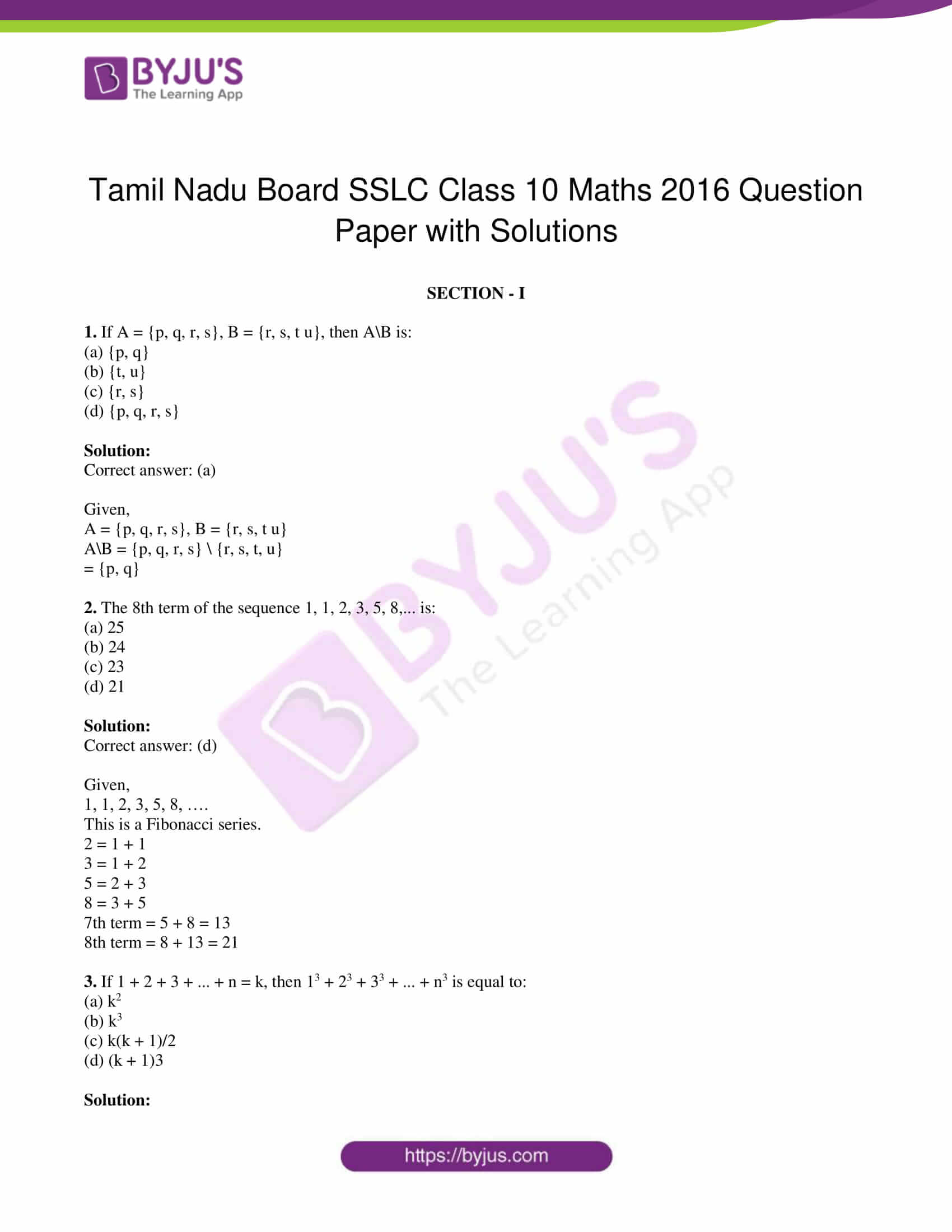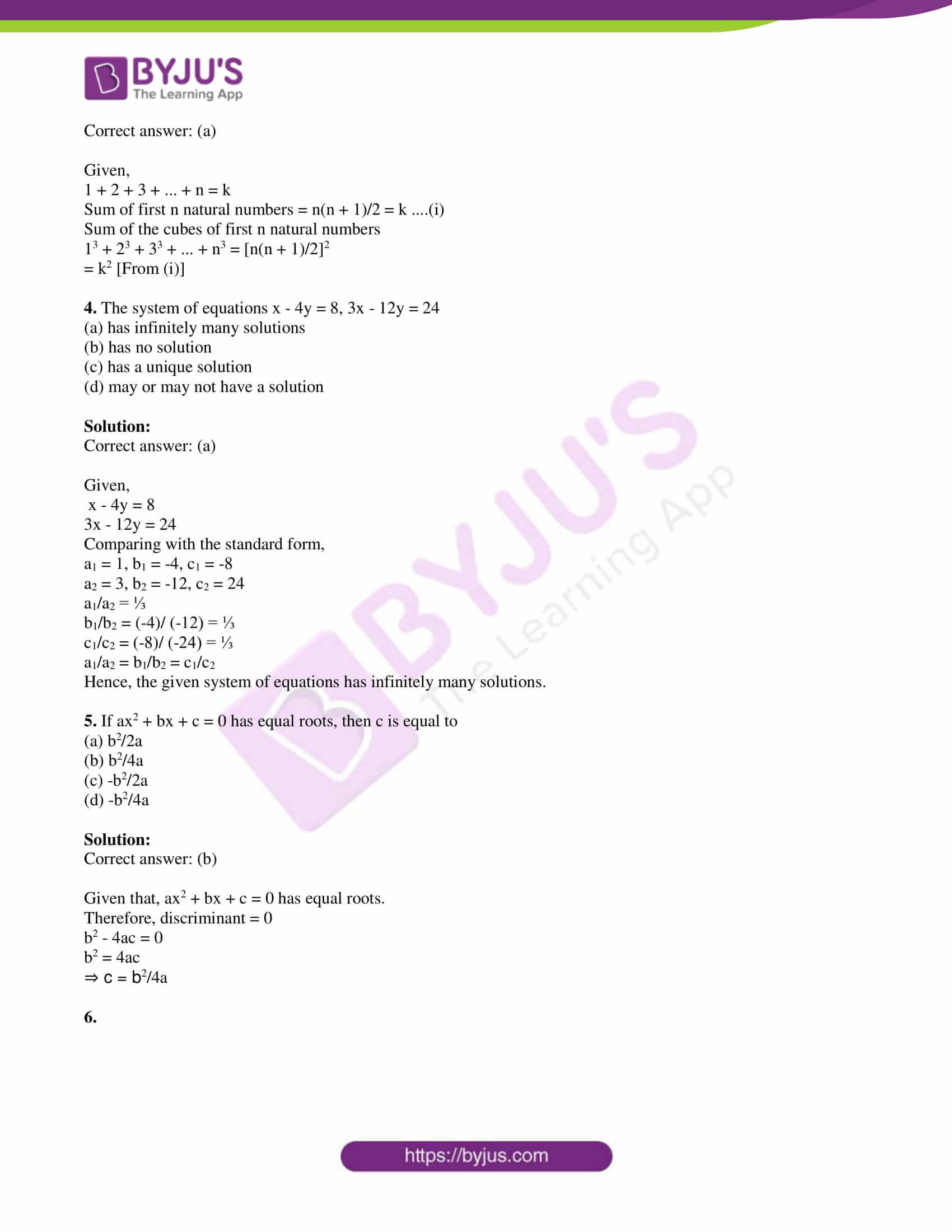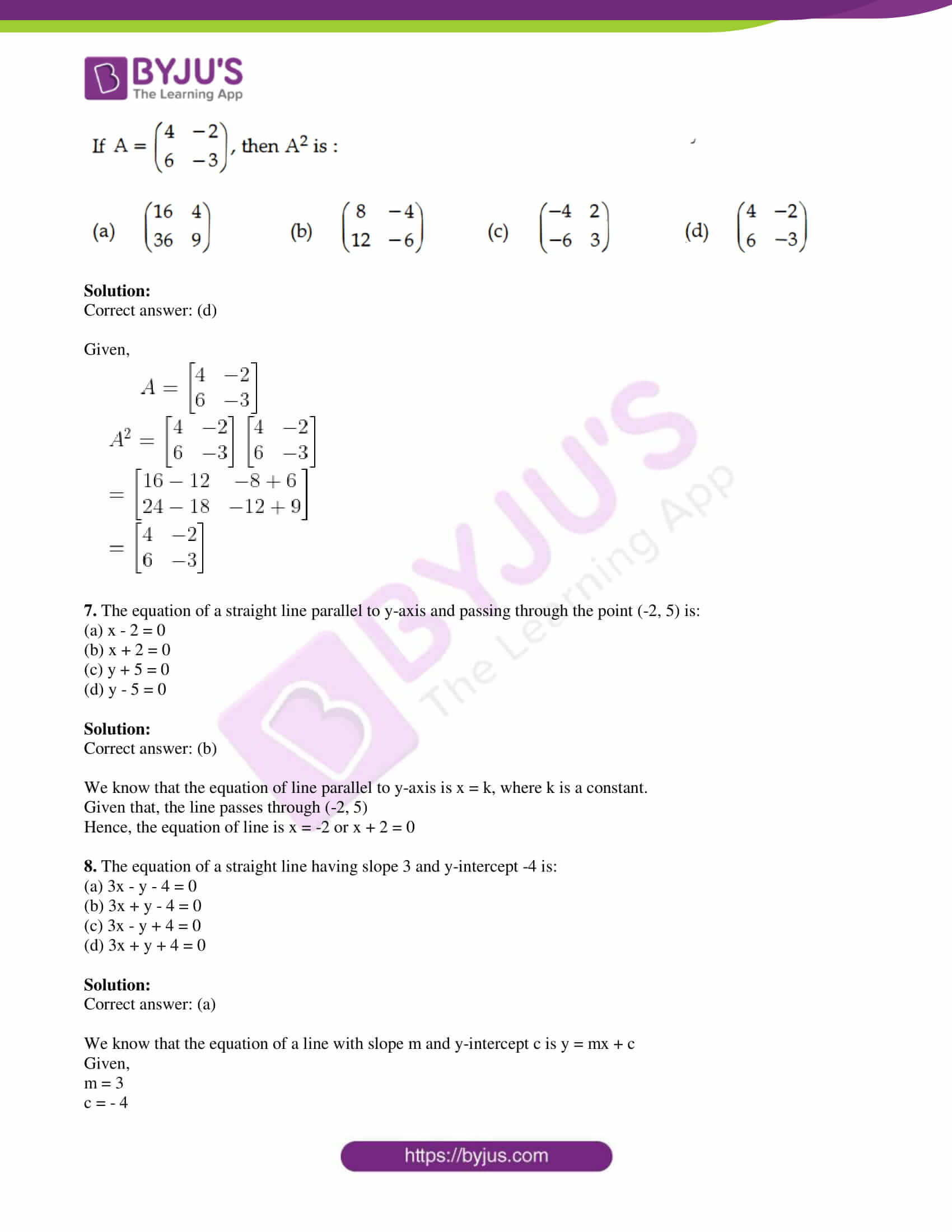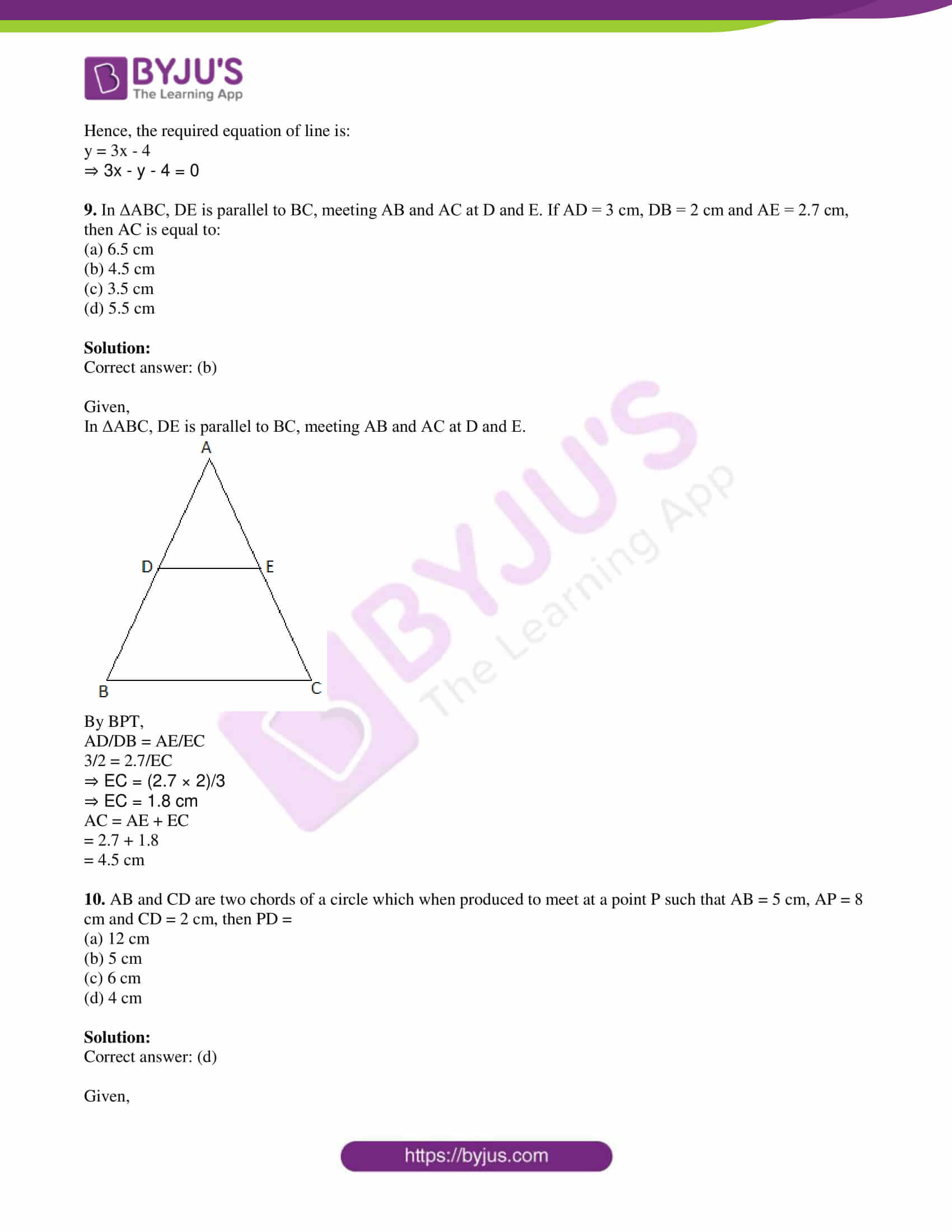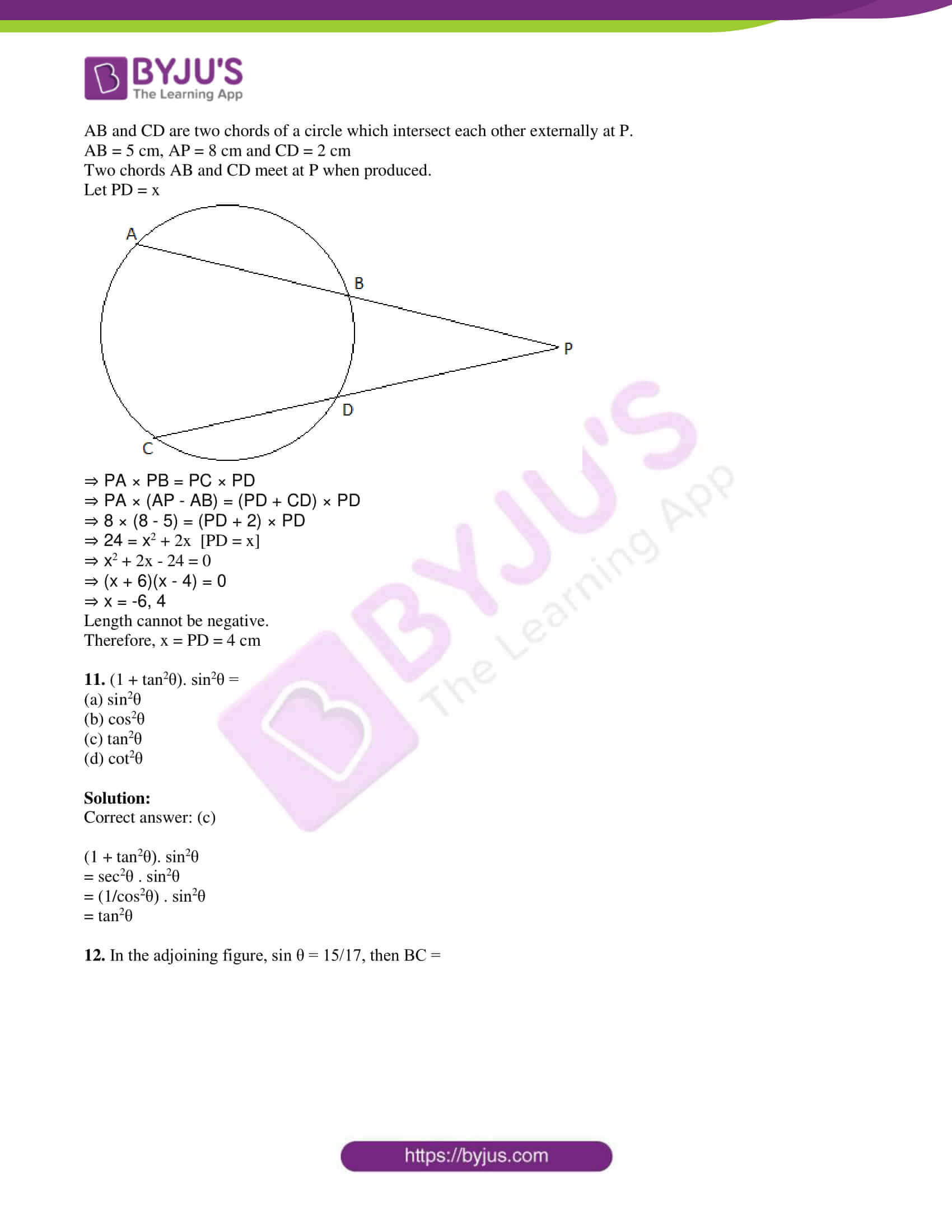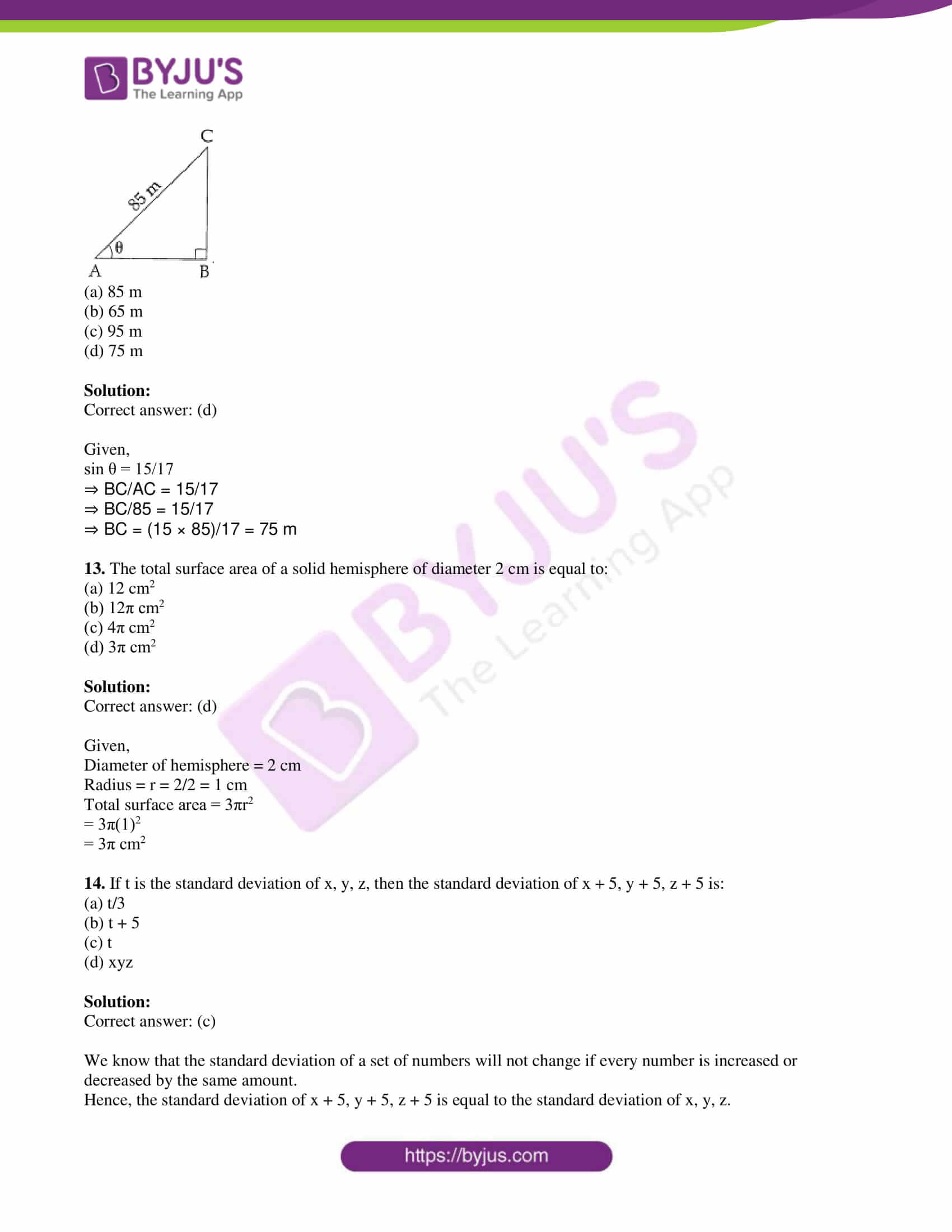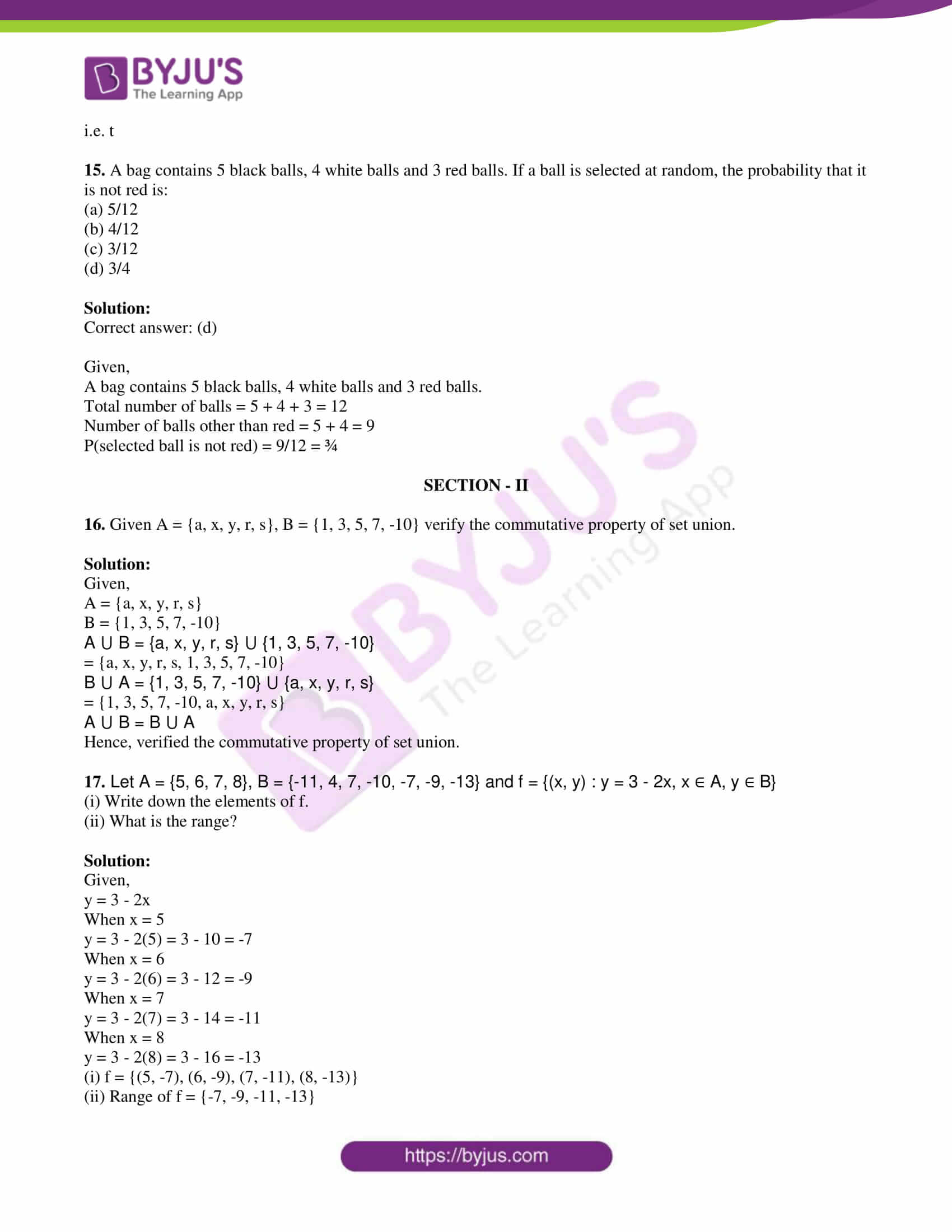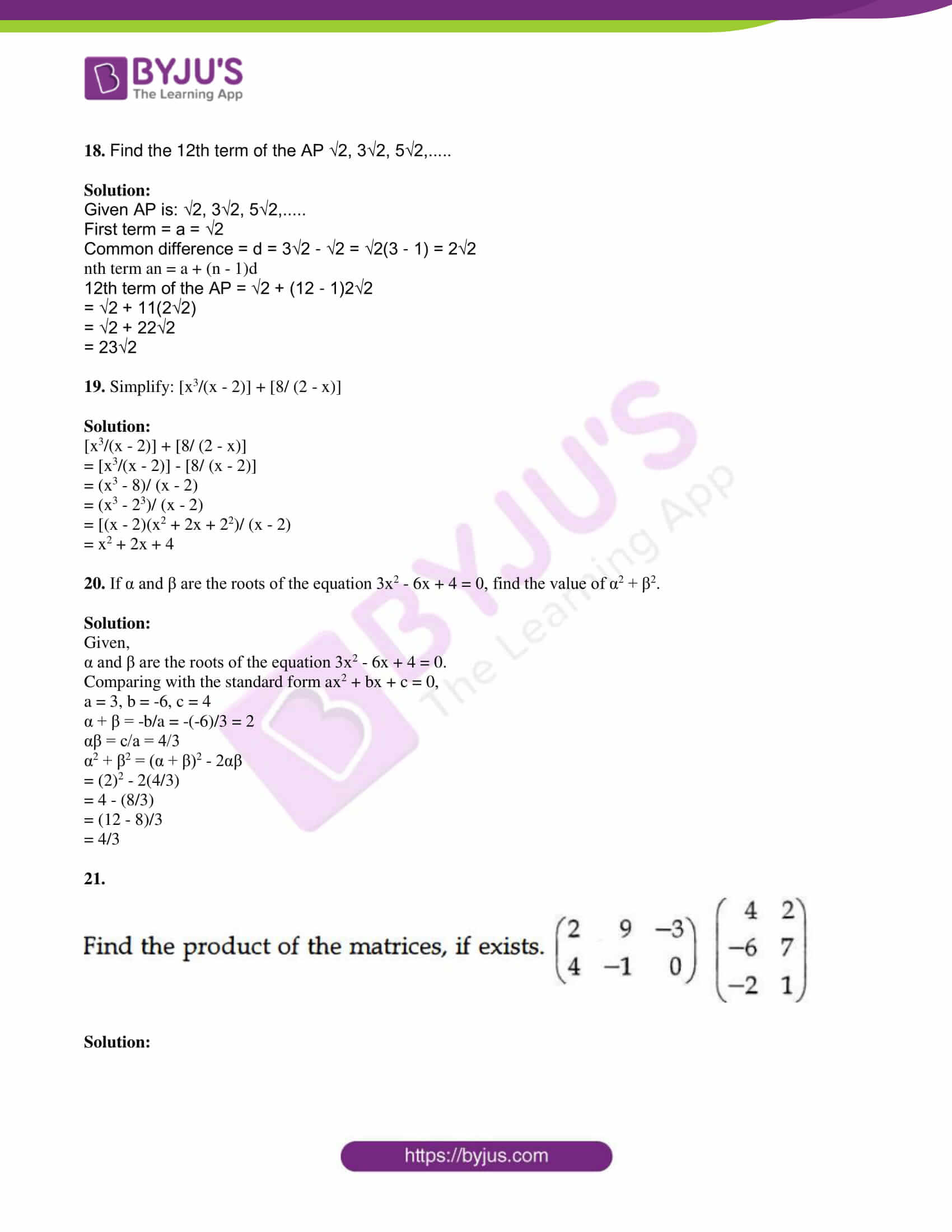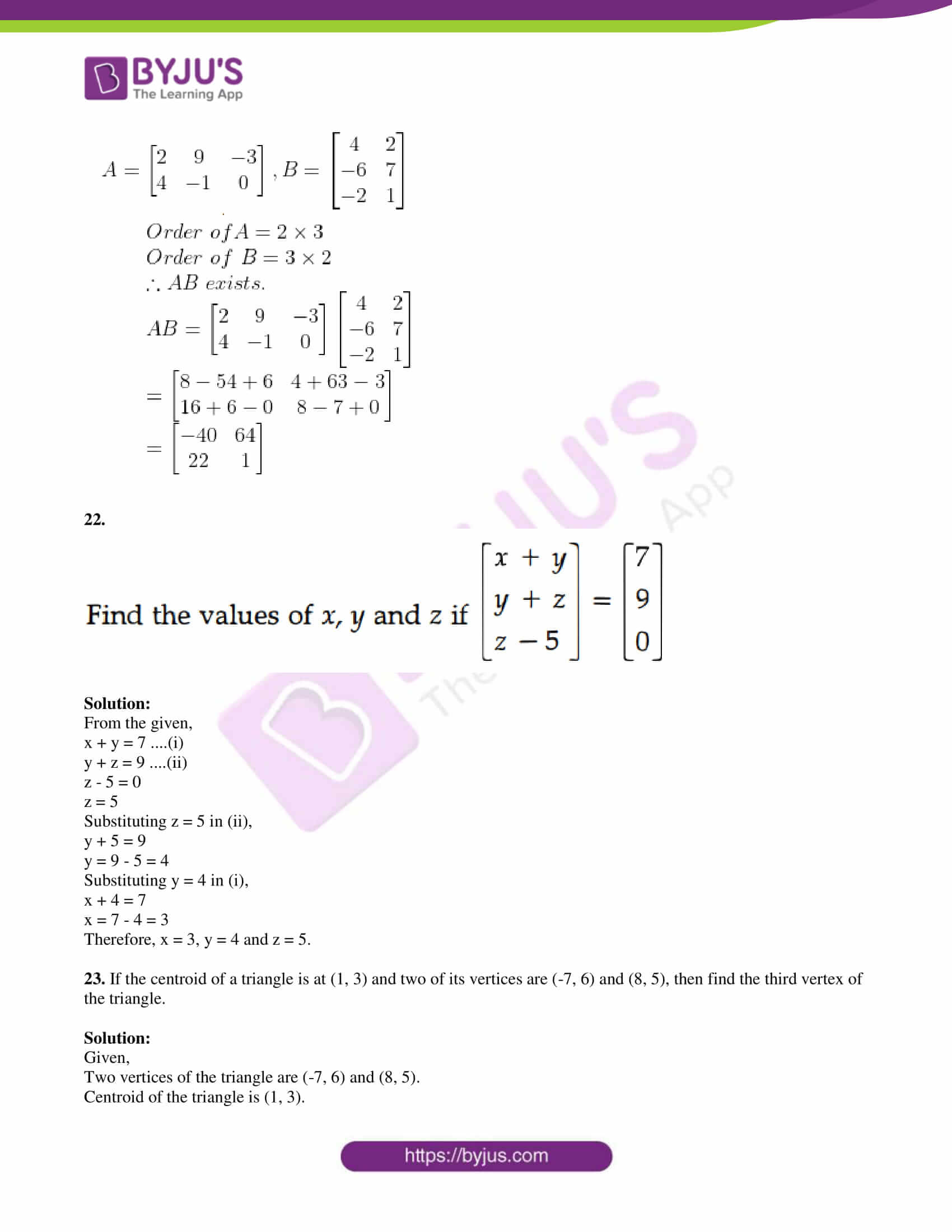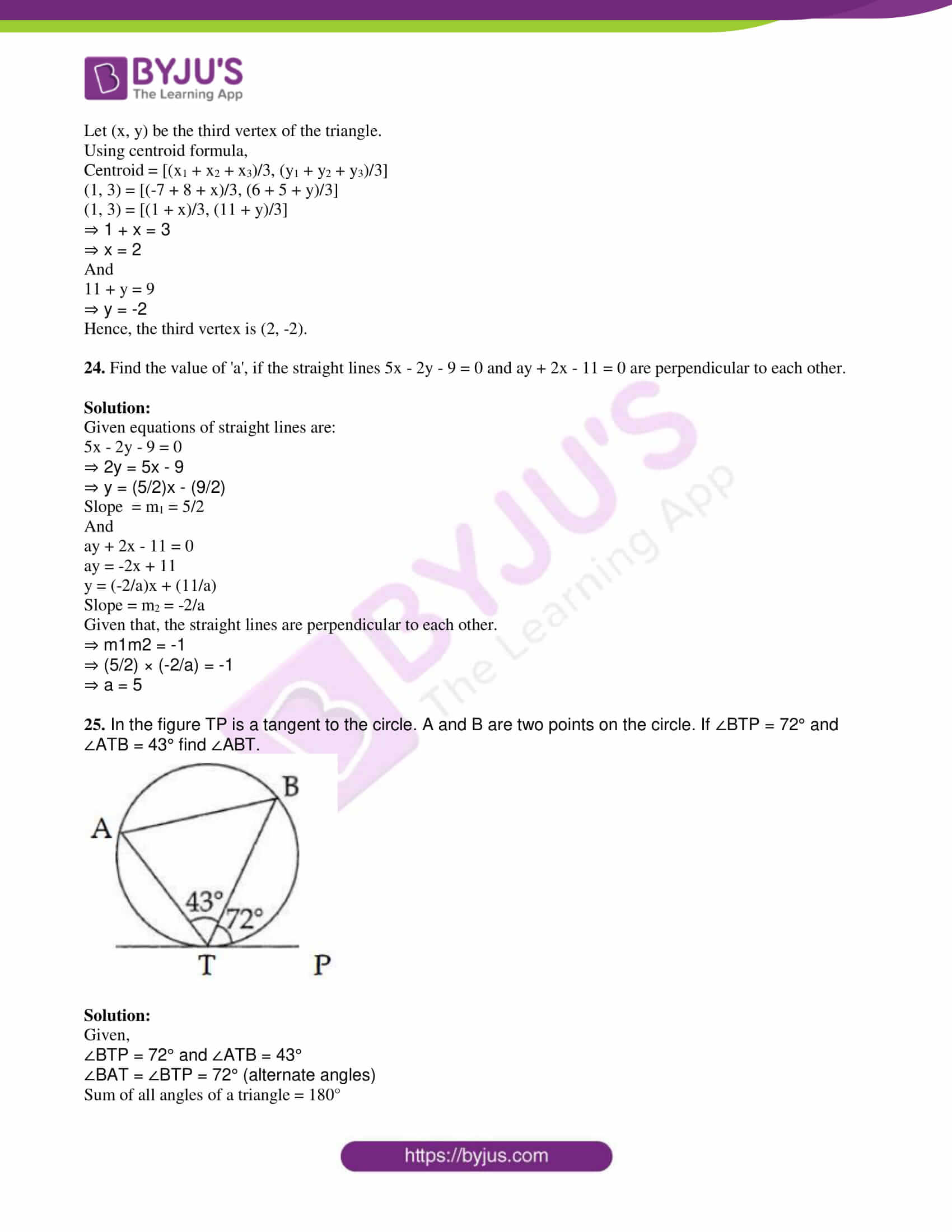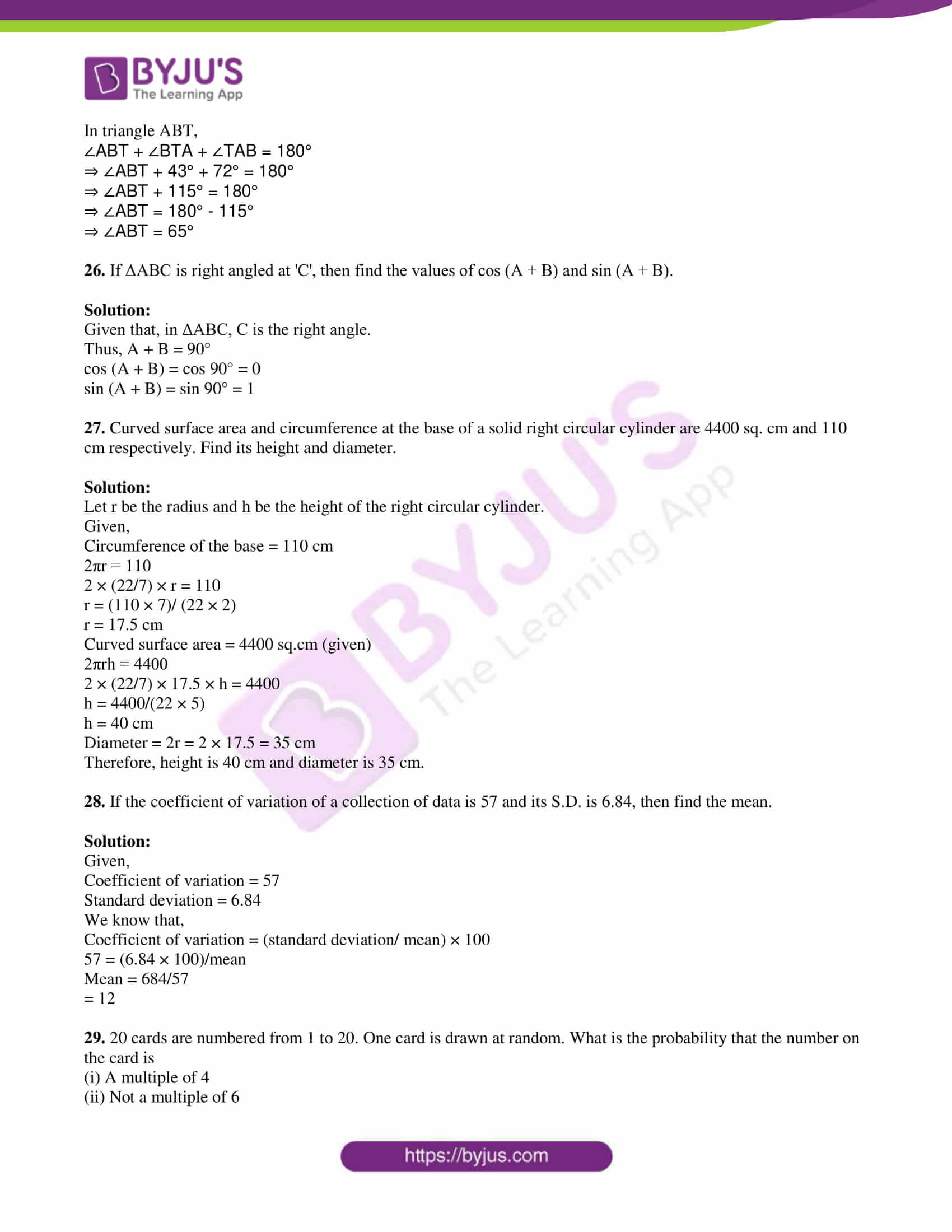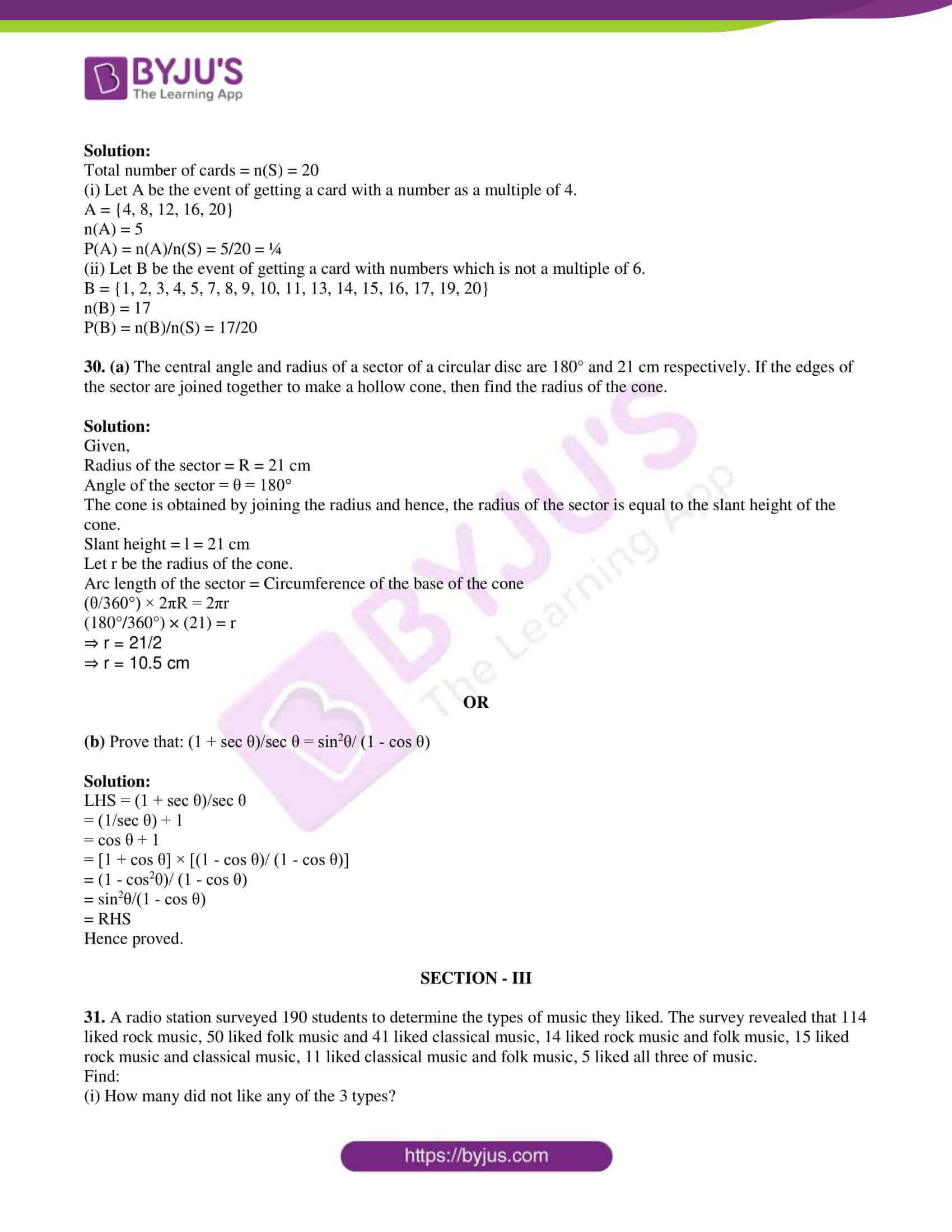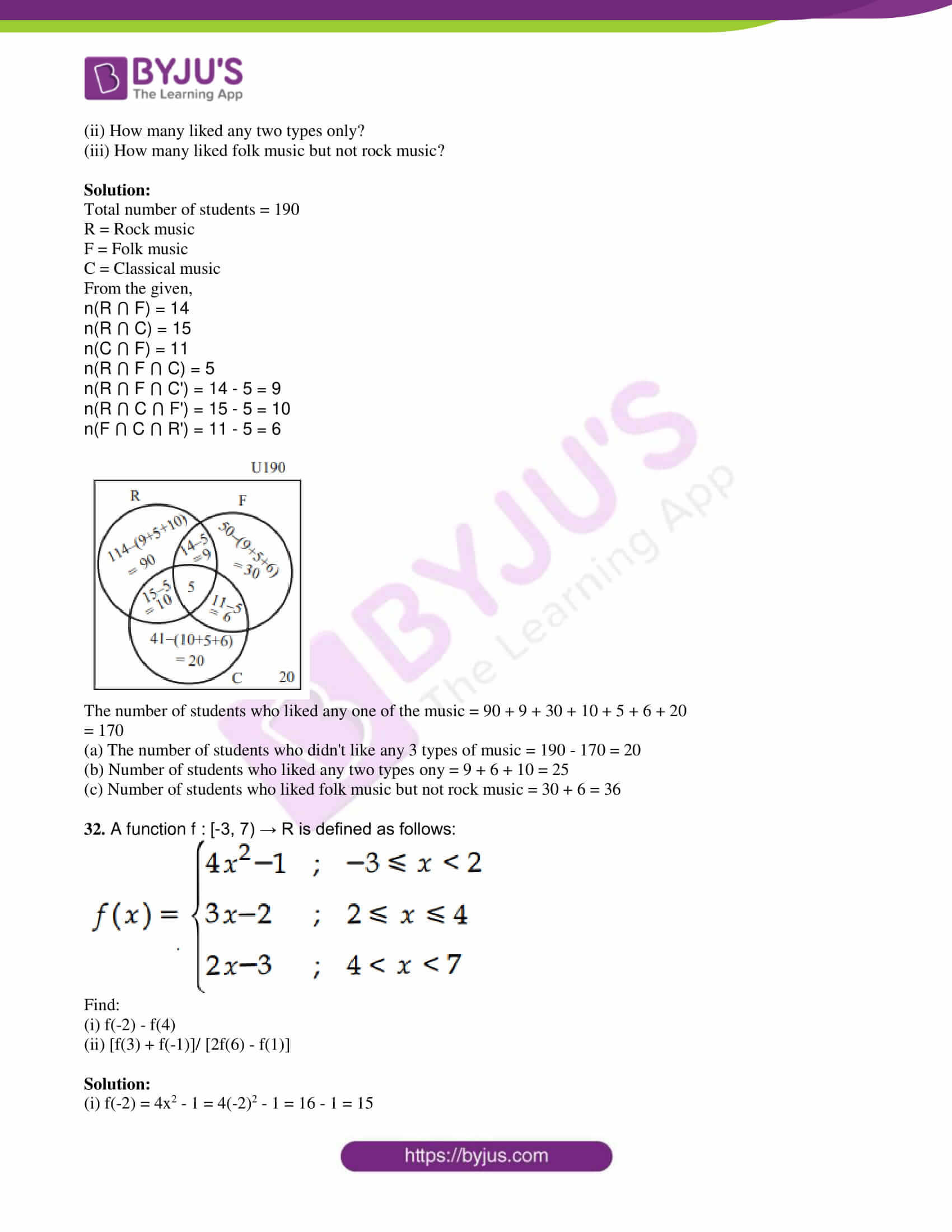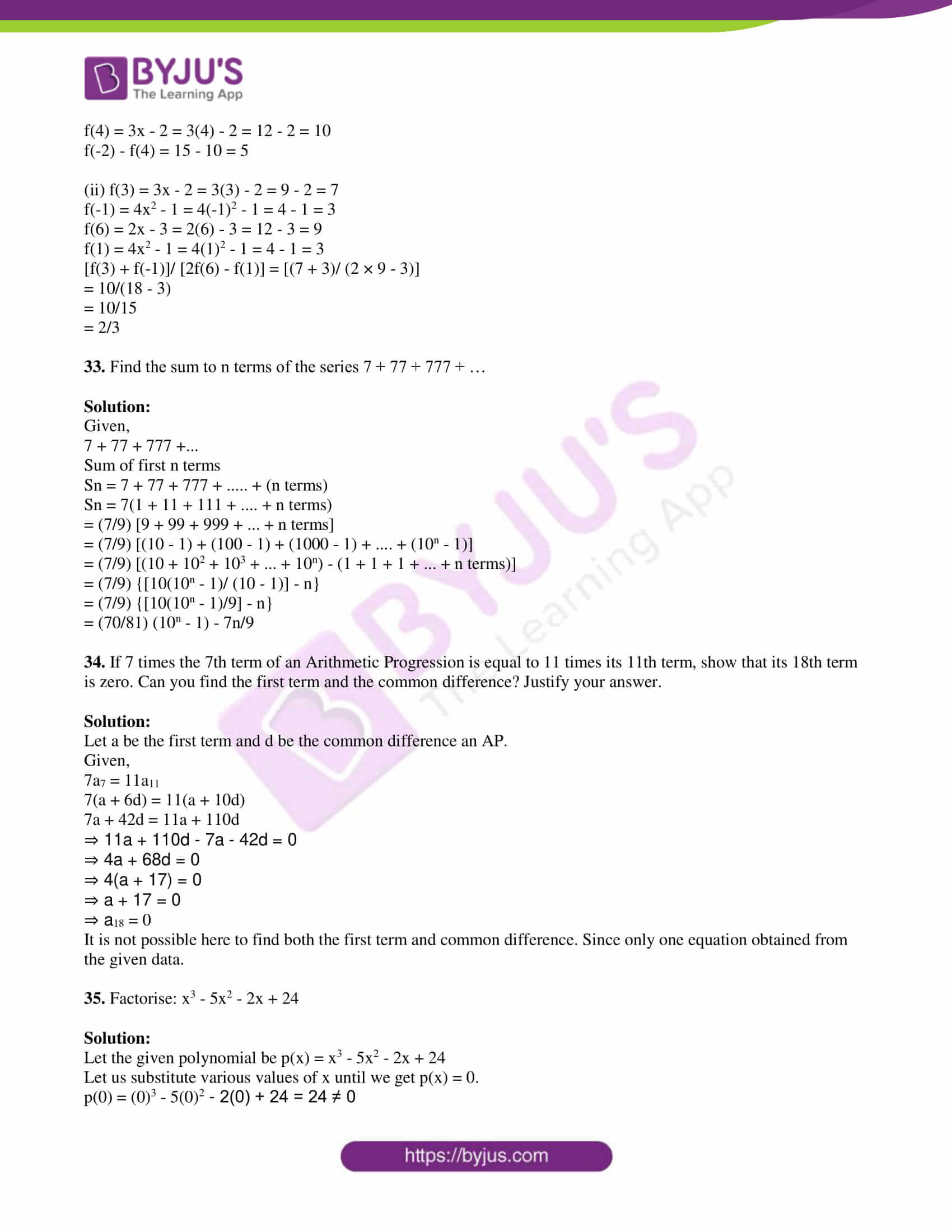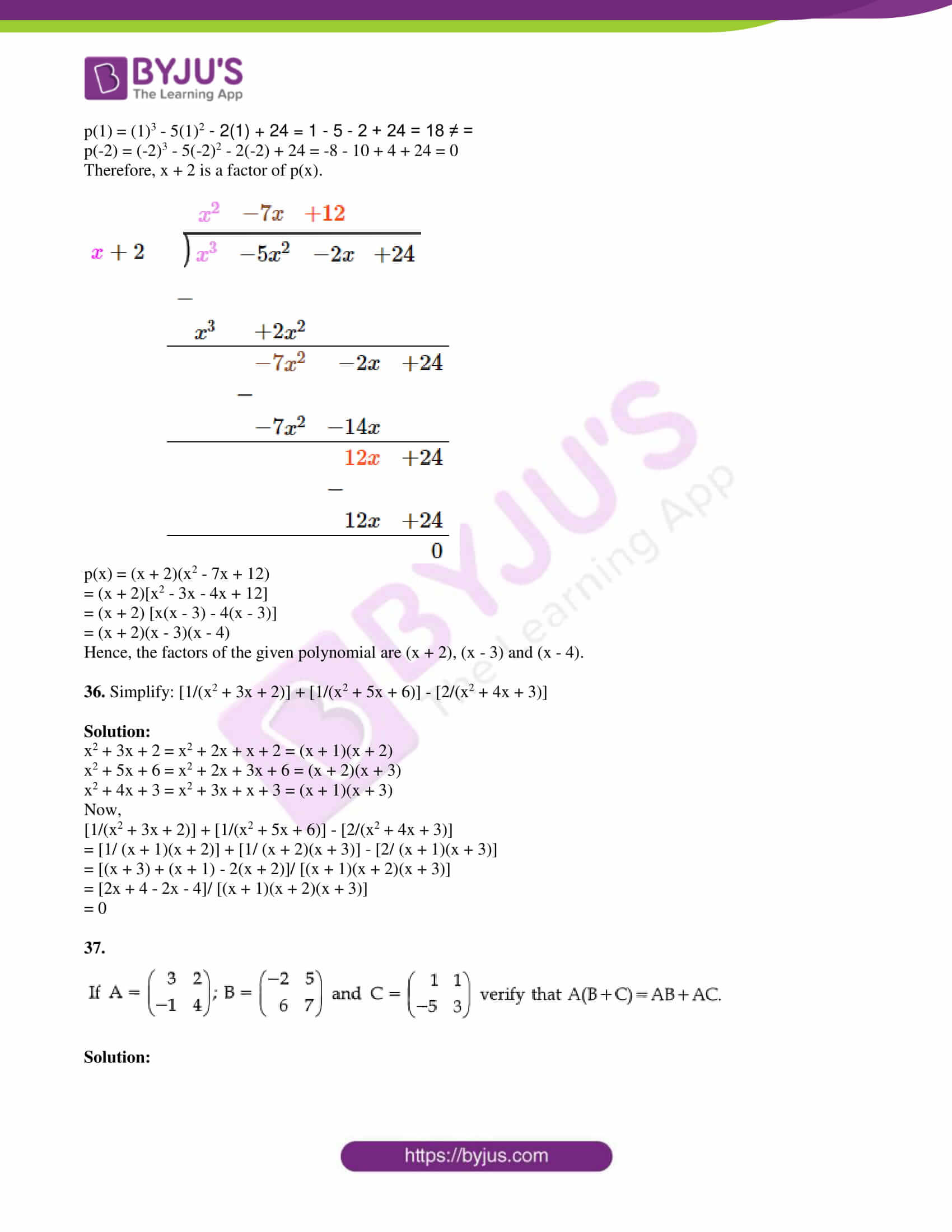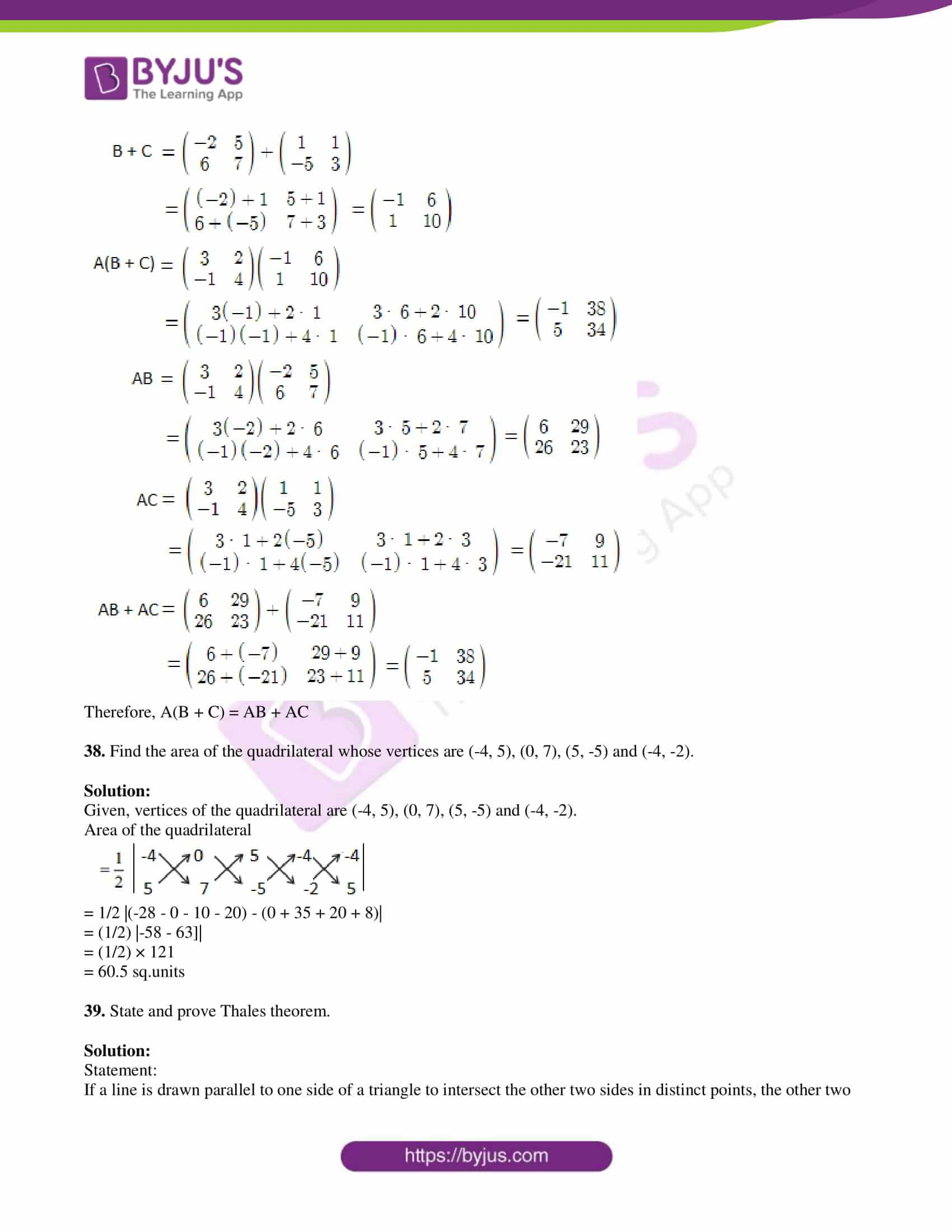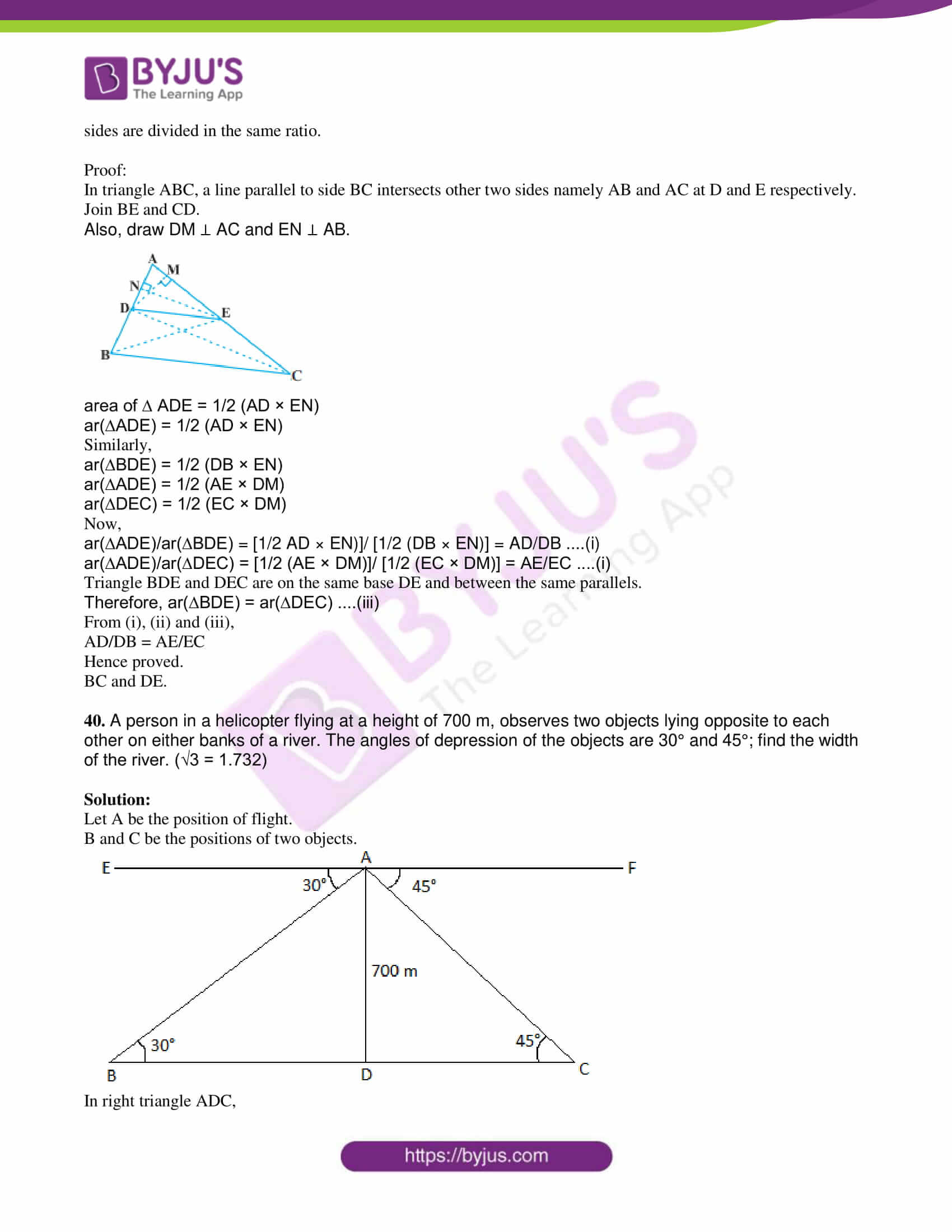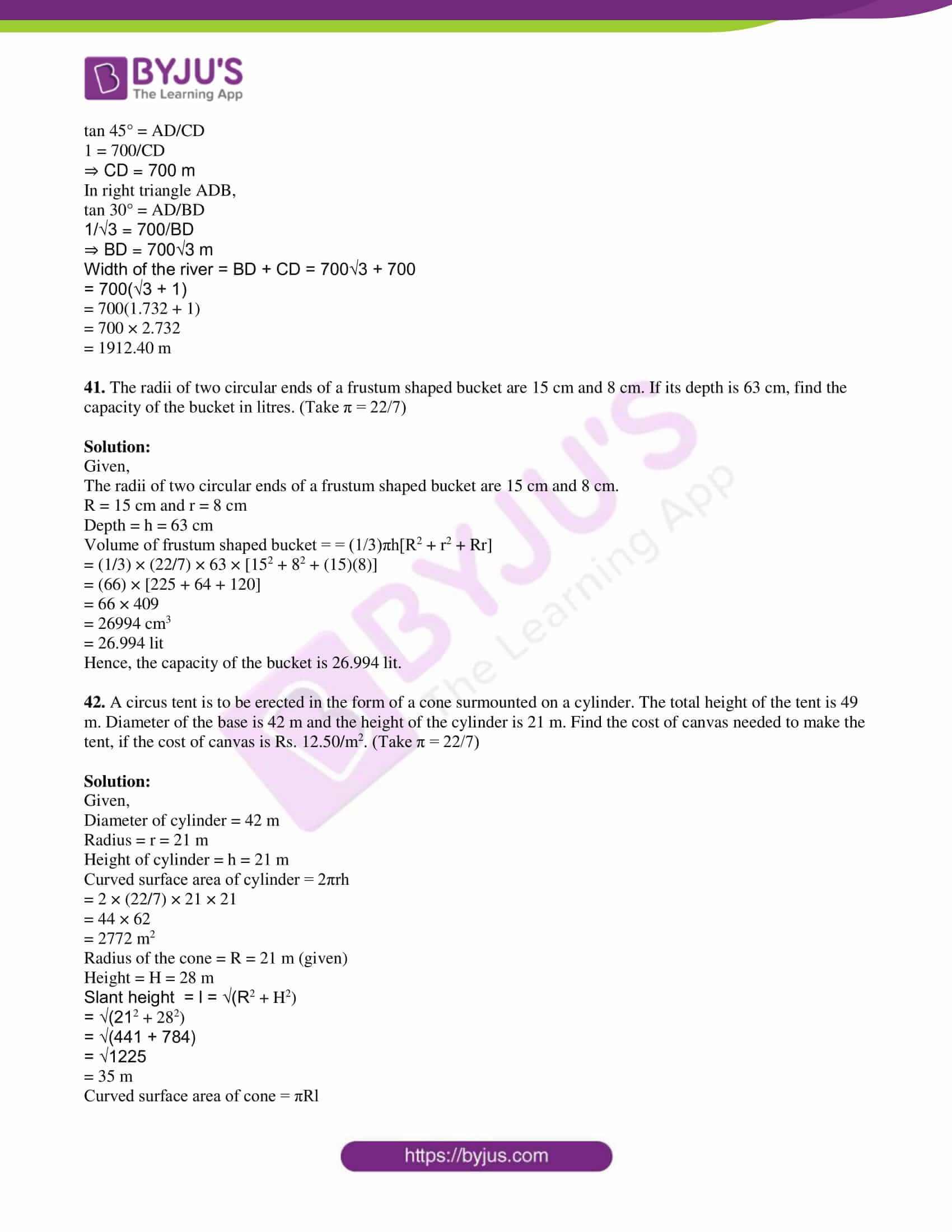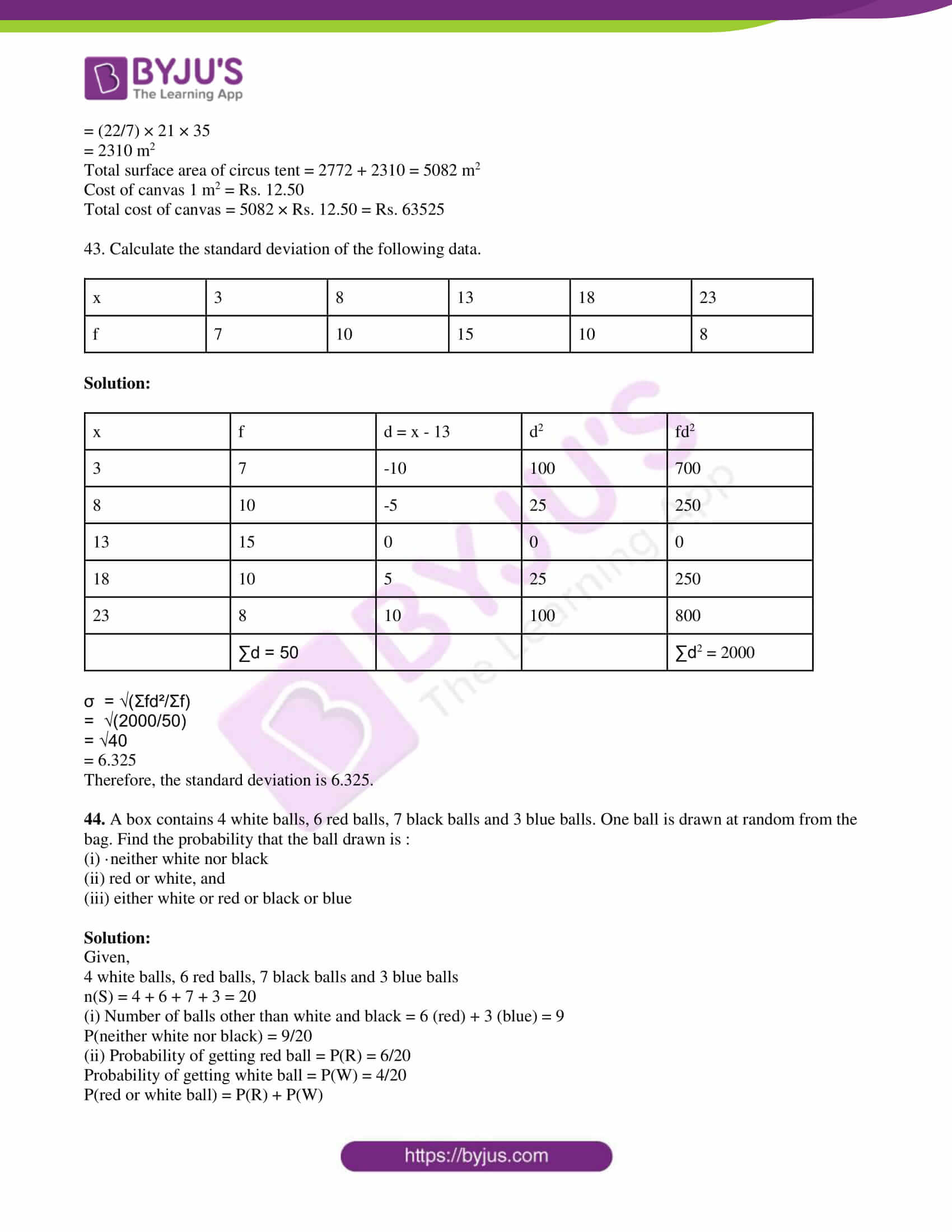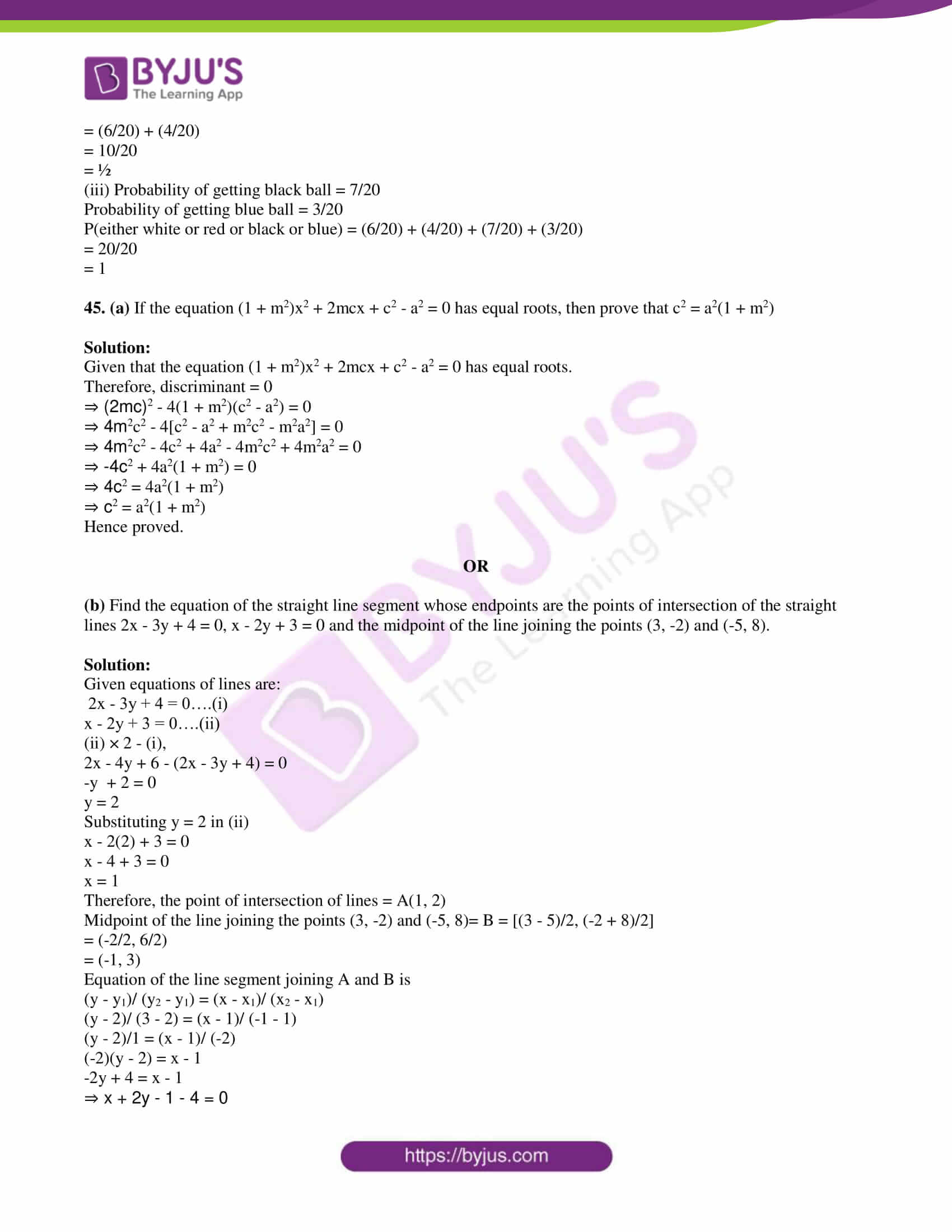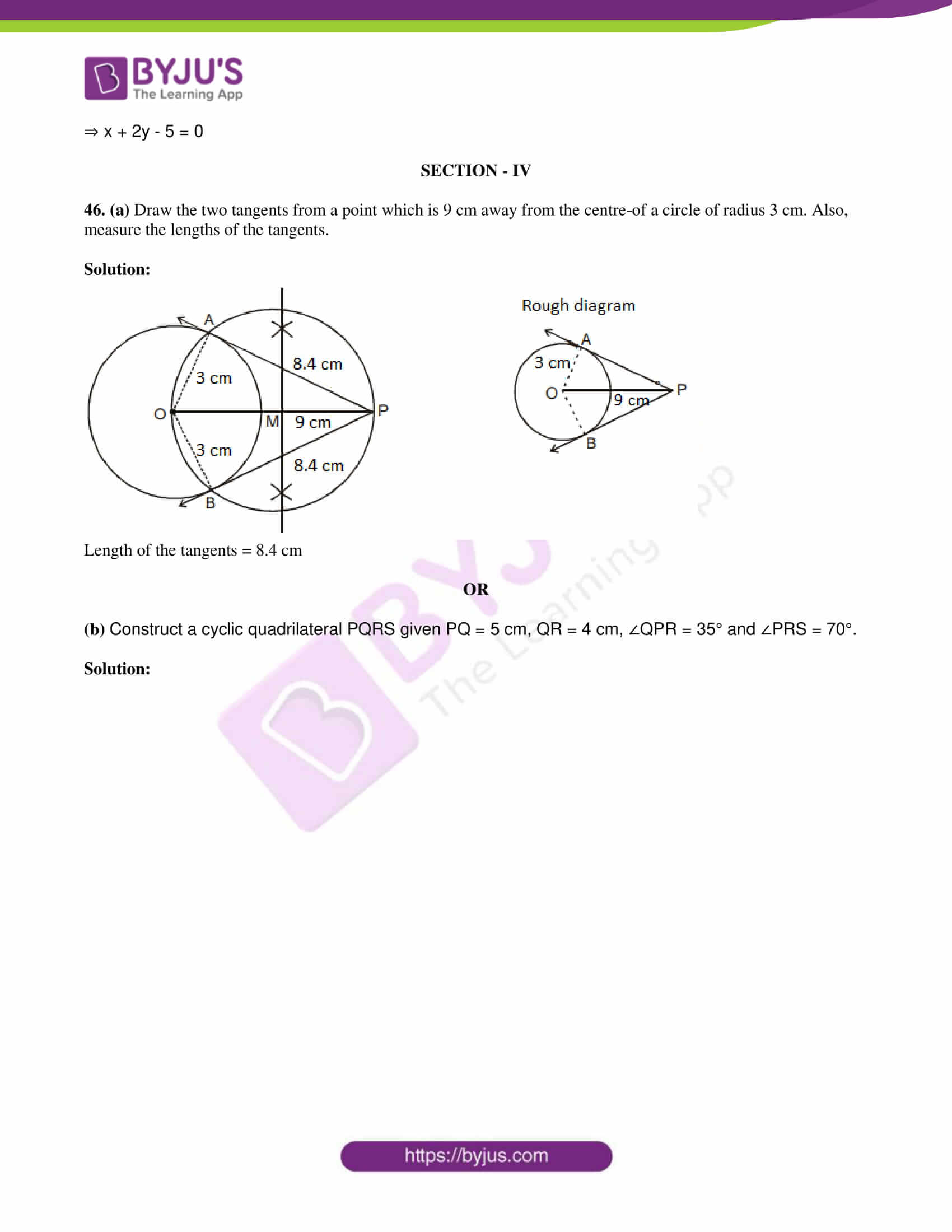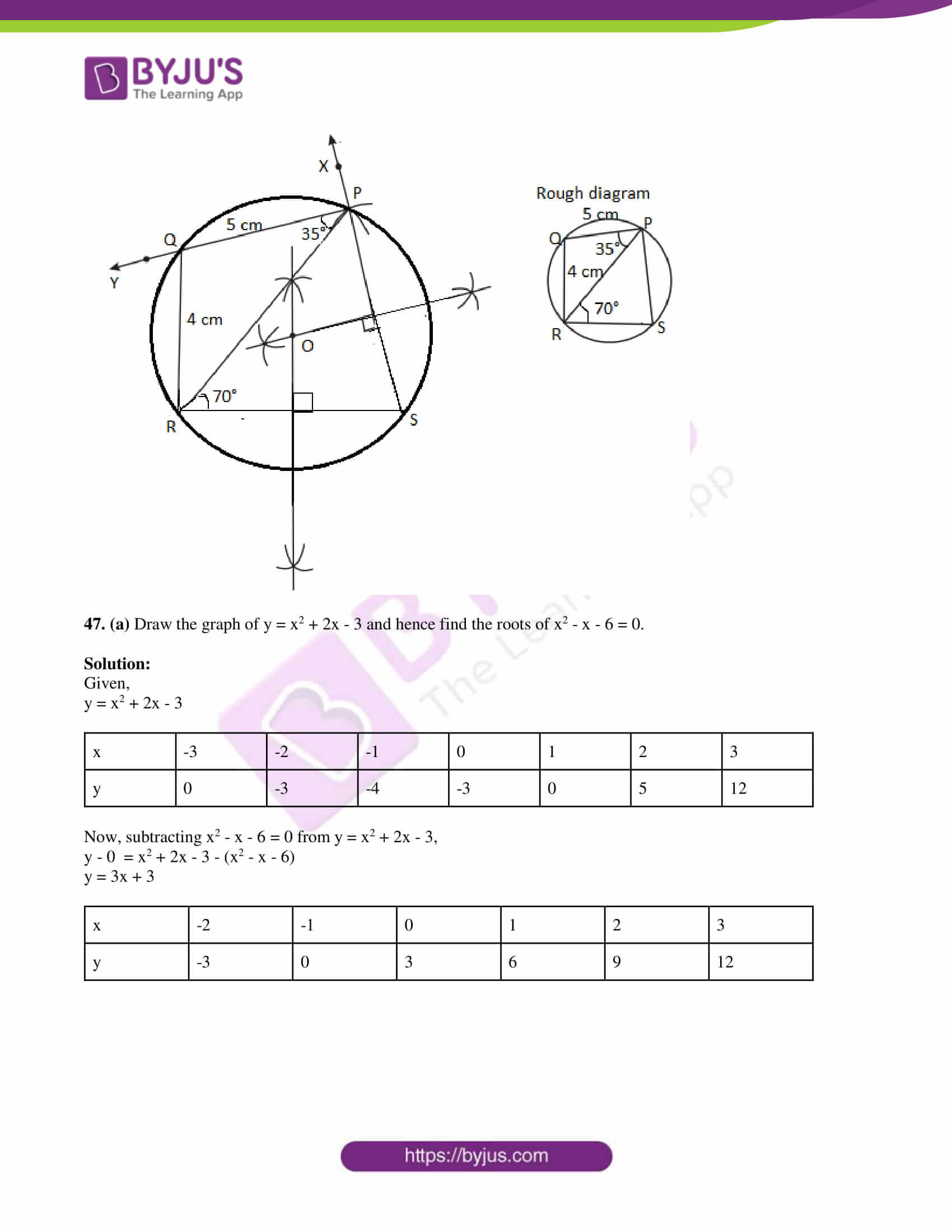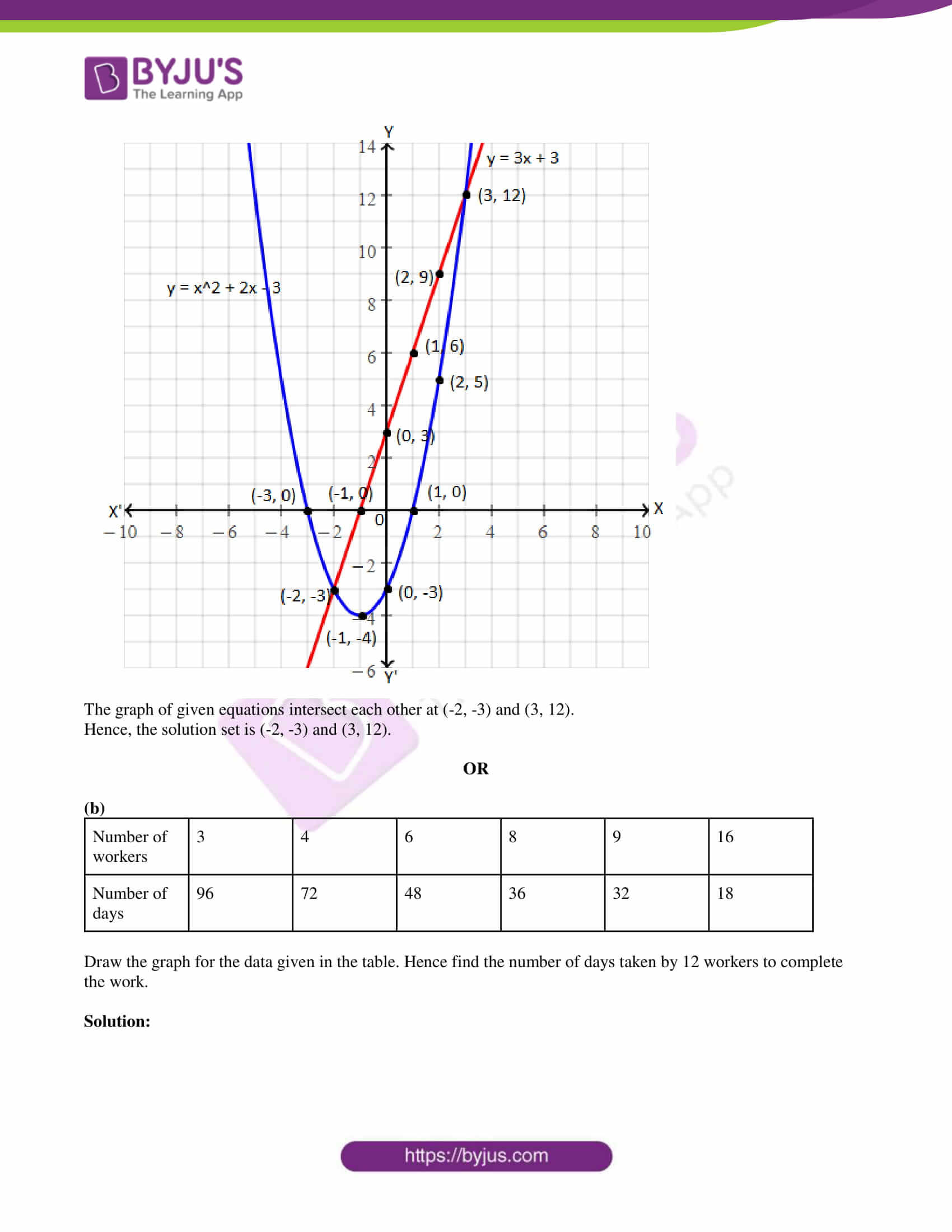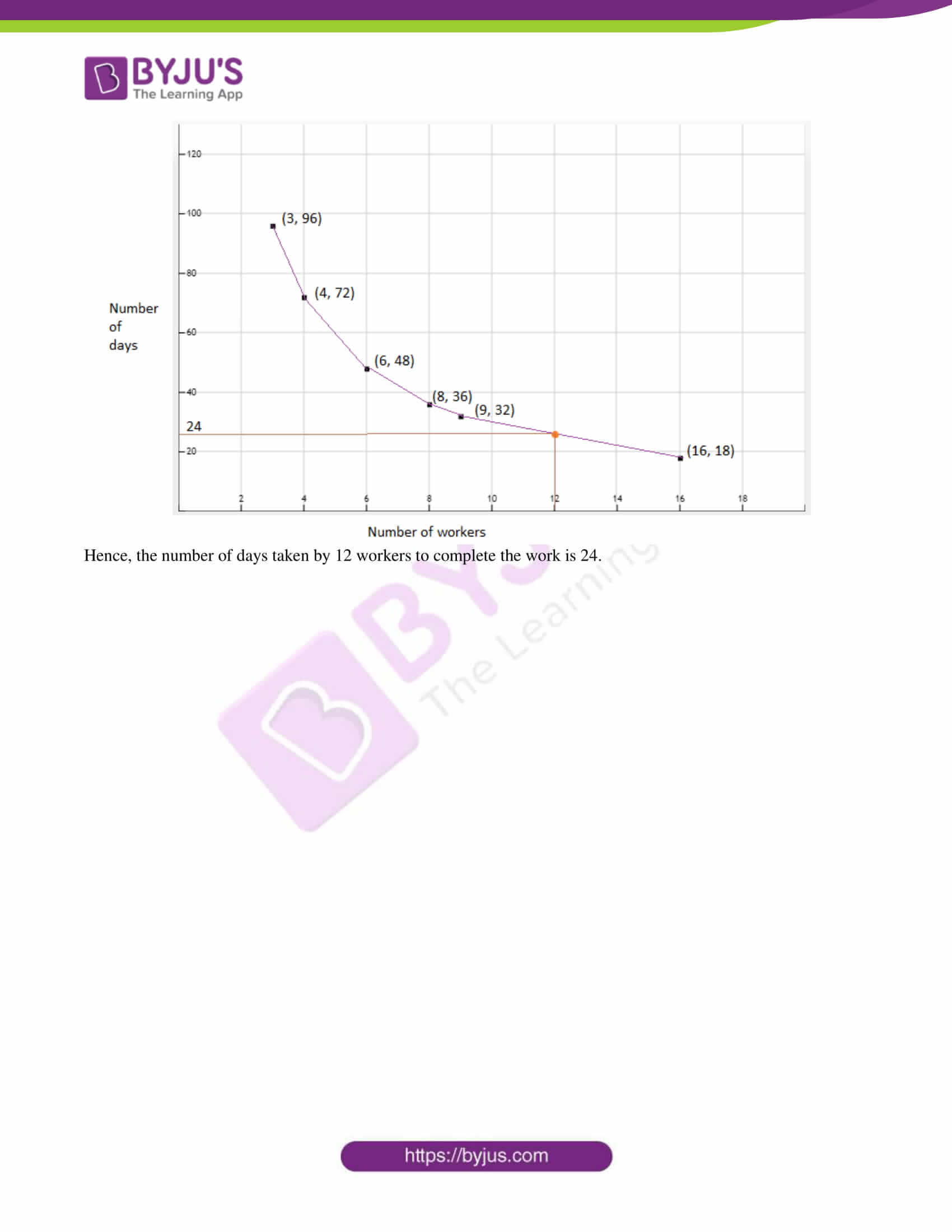SECTION – I

1. If A = {p, q, r, s}, B = {r, s, t u}, then A\B is:

(a) {p, q}

(b) {t, u}

(c) {r, s}

(d) {p, q, r, s}

Solution:

Given,

A = {p, q, r, s}, B = {r, s, t u}

A\B = {p, q, r, s} \ {r, s, t, u}

= {p, q}

2. The 8th term of the sequence 1, 1, 2, 3, 5, 8,… is:

(a) 25

(b) 24

(c) 23

(d) 21

Solution:

Given,

1, 1, 2, 3, 5, 8, ….

This is a fibonacci series.

2 = 1 + 1

3 = 1 + 2

5 = 2 + 3

8 = 3 + 5

7th term = 5 + 8 = 13

8th term = 8 + 13 = 21

3. If 1 + 2 + 3 + … + n = k, then 13 + 23 + 33 + … + n3 is equal to:

(a) k2

(b) k3

(c) k(k + 1)/2

(d) (k + 1)3

Solution:

Given,

1 + 2 + 3 + … + n = k

Sum of first n natural numbers = n(n + 1)/2 = k ….(i)

Sum of the cubes of first n natural numbers

13 + 23 + 33 + … + n3 = [n(n + 1)/2]2

= k2 [From (i)]

4. The system of equations x – 4y = 8, 3x – 12y = 24

(a) has infinitely many solutions

(b) has no solution

(c) has a unique solution

(d) may or may not have a solution

Solution:

Given,

x – 4y = 8

3x – 12y = 24

Comparing with the standard form,

a1 = 1, b1 = -4, c1 = -8

a2 = 3, b2 = -12, c2 = 24

a1/a2 = ⅓

b1/b2 = (-4)/ (-12) = ⅓

c1/c2 = (-8)/ (-24) = ⅓

a1/a2 = b1/b2 = c1/c2

Hence, the given system of equations has infinitely many solutions.

5. If ax2 + bx + c = 0 has equal roots, then c is equal to

(a) b2/2a

(b) b2/4a

(c) -b2/2a

(d) -b2/4a

Solution:

Given that, ax2 + bx + c = 0 has equal roots.

Therefore, discriminant = 0

b2 – 4ac = 0

b2 = 4ac

⇒ c = b2/4a

6.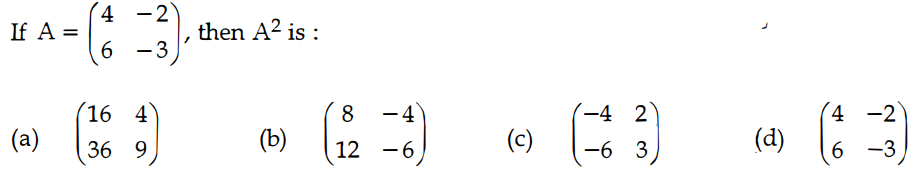Solution:

Given,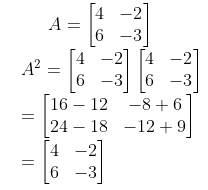7. The equation of a straight line parallel to y-axis and passing through the point (-2, 5) is:

(a) x – 2 = 0

(b) x + 2 = 0

(c) y + 5 = 0

(d) y – 5 = 0

Solution:

We know that the equation of line parallel to y-axis is x = k, where k is a constant.

Given that, the line passes through (-2, 5)

Hence, the equation of line is x = -2 or x + 2 = 0

8. The equation of a straight line having slope 3 and y-intercept -4 is:

(a) 3x – y – 4 = 0

(b) 3x + y – 4 = 0

(c) 3x – y + 4 = 0

(d) 3x + y + 4 = 0

Solution:

We know that the equation of a line with slope m and y-intercept c is y = mx + c

Given,

m = 3

c = – 4

Hence, the required equation of line is:

y = 3x – 4

⇒ 3x – y – 4 = 0

9. In ΔABC, DE is parallel to BC, meeting AB and AC at D and E. If AD = 3 cm, DB = 2 cm and AE = 2.7 cm, then AC is equal to:

(a) 6.5 cm

(b) 4.5 cm

(c) 3.5 cm

(d) 5.5 cm

Solution:

Given,

In ΔABC, DE is parallel to BC, meeting AB and AC at D and E.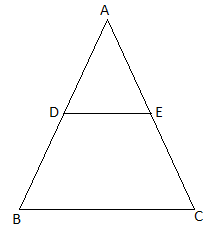By BPT,

3/2 = 2.7/EC

⇒ EC = (2.7 × 2)/3

⇒ EC = 1.8 cm

AC = AE + EC

= 2.7 + 1.8

= 4.5 cm

10. AB and CD are two chords of a circle which when produced to meet at a point P such that AB = 5 cm, AP = 8 cm and CD = 2 cm, then PD =

(a) 12 cm

(b) 5 cm

(c) 6 cm

(d) 4 cm

Solution:

Given,

AB and CD are two chords of a circle which intersect each other externally at P.

AB = 5 cm, AP = 8 cm and CD = 2 cm

Two chords AB and CD meet at P when produced.

Let PD = x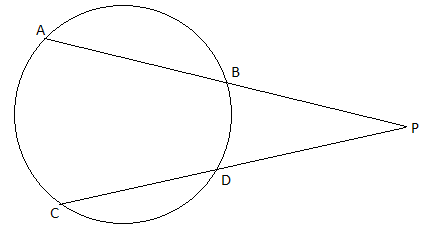⇒ PA × PB = PC × PD

⇒ PA × (AP – AB) = (PD + CD) × PD

⇒ 8 × (8 – 5) = (PD + 2) × PD

⇒ 24 = x2 + 2x [PD = x]

⇒ x2 + 2x – 24 = 0

⇒ (x + 6)(x – 4) = 0

⇒ x = -6, 4

Length cannot be negative.

Therefore, x = PD = 4 cm

11. (1 + tan2θ). sin2θ =

(a) sin2θ

(b) cos2θ

(c) tan2θ

(d) cot2θ

Solution:

(1 + tan2θ). sin2θ

= sec2θ . sin2θ

= (1/cos2θ) . sin2θ

= tan2θ

12. In the adjoining figure, sin θ = 15/17, then BC =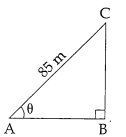(a) 85 m

(b) 65 m

(c) 95 m

(d) 75 m

Solution:

Given,

sin θ = 15/17

⇒ BC/AC = 15/17

⇒ BC/85 = 15/17

⇒ BC = (15 × 85)/17 = 75 m

13. The total surface area of a solid hemisphere of diameter 2 cm is equal to:

(a) 12 cm2

(b) 12π cm2

(c) 4π cm2

(d) 3π cm2

Solution:

Given,

Diameter of hemisphere = 2 cm

Radius = r = 2/2 = 1 cm

Total surface area = 3πr2

= 3π(1)2

= 3π cm2

14. If t is the standard deviation of x, y, z, then the standard deviation of x + 5, y + 5, z + 5 is:

(a) t/3

(b) t + 5

(c) t

(d) xyz

Solution:

We know that the standard deviation of a set of numbers will not change if every number is increased or decreased by the same amount.

Hence, the standard deviation of x + 5, y + 5, z + 5 is equal to the standard deviation of x, y, z.

i.e. t

15. A bag contains 5 black balls, 4 white balls and 3 red balls. If a ball is selected at random, the probability that it is not red is:

(a) 5/12

(b) 4/12

(c) 3/12

(d) 3/4

Solution:

Given,

A bag contains 5 black balls, 4 white balls and 3 red balls.

Total number of balls = 5 + 4 + 3 = 12

Number of balls other than red = 5 + 4 = 9

P(selected ball is not red) = 9/12 = ¾

SECTION – II

16. Given A = {a, x, y, r, s}, B = {1, 3, 5, 7, -10} verify the commutative property of set union.

Solution:

Given,

A = {a, x, y, r, s}

B = {1, 3, 5, 7, -10}

A ⋃ B = {a, x, y, r, s} ⋃ {1, 3, 5, 7, -10}

= {a, x, y, r, s, 1, 3, 5, 7, -10}

B ⋃ A = {1, 3, 5, 7, -10} ⋃ {a, x, y, r, s}

= {1, 3, 5, 7, -10, a, x, y, r, s}

A ⋃ B = B ⋃ A

Hence, verified the commutative property of set union.

17. Let A = {5, 6, 7, 8}, B = {-11, 4, 7, -10, -7, -9, -13} and f = {(x, y) : y = 3 – 2x, x ∈ A, y ∈ B}

(i) Write down the elements of f.

(ii) What is the range?

Solution:

Given,

y = 3 – 2x

When x = 5

y = 3 – 2(5) = 3 – 10 = -7

When x = 6

y = 3 – 2(6) = 3 – 12 = -9

When x = 7

y = 3 – 2(7) = 3 – 14 = -11

When x = 8

y = 3 – 2(8) = 3 – 16 = -13

(i) f = {(5, -7), (6, -9), (7, -11), (8, -13)}

(ii) Range of f = {-7, -9, -11, -13}

18. Find the 12th term of the AP √2, 3√2, 5√2,…..

Solution:

Given AP is: √2, 3√2, 5√2,…..

First term = a = √2

Common difference = d = 3√2 – √2 = √2(3 – 1) = 2√2

nth term an = a + (n – 1)d

12th term of the AP = √2 + (12 – 1)2√2

= √2 + 11(2√2)

= √2 + 22√2

= 23√2

19. Simplify: [x3/(x – 2)] + [8/ (2 – x)]

Solution:

[x3/(x – 2)] + [8/ (2 – x)]

= [x3/(x – 2)] – [8/ (x – 2)]

= (x3 – 8)/ (x – 2)

= (x3 – 23)/ (x – 2)

= [(x – 2)(x2 + 2x + 22)/ (x – 2)

= x2 + 2x + 4

20. If α and β are the roots of the equation 3x2 – 6x + 4 = 0, find the value of α2 + β2.

Solution:

Given,

α and β are the roots of the equation 3x2 – 6x + 4 = 0.

Comparing with the standard form ax2 + bx + c = 0,

a = 3, b = -6, c = 4

α + β = -b/a = -(-6)/3 = 2

αβ = c/a = 4/3

α2 + β2 = (α + β)2 – 2αβ

= (2)2 – 2(4/3)

= 4 – (8/3)

= (12 – 8)/3

= 4/3

21.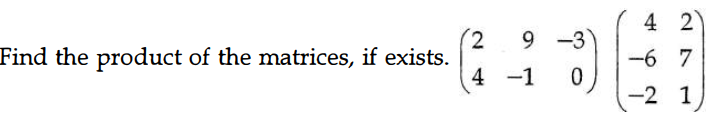Solution: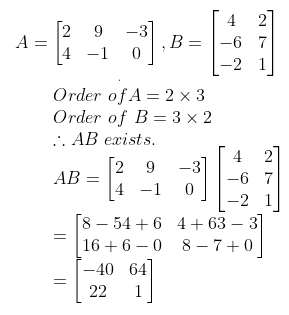22.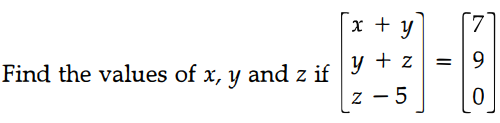Solution:

From the given,

x + y = 7 ….(i)

y + z = 9 ….(ii)

z – 5 = 0

z = 5

Substituting z = 5 in (ii),

y + 5 = 9

y = 9 – 5 = 4

Substituting y = 4 in (i),

x + 4 = 7

x = 7 – 4 = 3

Therefore, x = 3, y = 4 and z = 5.

23. If the centroid of a triangle is at (1, 3) and two of its vertices are (-7, 6) and (8, 5), then find the third vertex of the triangle.

Solution:

Given,

Two vertices of the triangle are (-7, 6) and (8, 5).

Centroid of the triangle is (1, 3).

Let (x, y) be the third vertex of the triangle.

Using centroid formula,

Centroid = [(x1 + x2 + x3)/3, (y1 + y2 + y3)/3]

(1, 3) = [(-7 + 8 + x)/3, (6 + 5 + y)/3]

(1, 3) = [(1 + x)/3, (11 + y)/3]

⇒ 1 + x = 3

⇒ x = 2

And

11 + y = 9

⇒ y = -2

Hence, the third vertex is (2, -2).

24. Find the value of ‘a’, if the straight lines 5x – 2y – 9 = 0 and ay + 2x – 11 = 0 are perpendicular to each other.

Solution:

Given equations of straight lines are:

5x – 2y – 9 = 0

⇒ 2y = 5x – 9

⇒ y = (5/2)x – (9/2)

Slope = m1 = 5/2

And

ay + 2x – 11 = 0

ay = -2x + 11

y = (-2/a)x + (11/a)

Slope = m2 = -2/a

Given that, the straight lines are perpendicular to each other.

⇒ m1m2 = -1

⇒ (5/2) × (-2/a) = -1

⇒ a = 5

25. In the figure TP is a tangent to the circle. A and B are two points on the circle. If ∠BTP = 72° and ∠ATB = 43° find ∠ABT.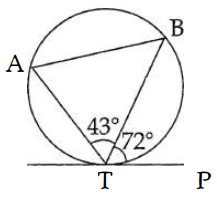Solution:

Given,

∠BTP = 72° and ∠ATB = 43°

∠BAT = ∠BTP = 72° (alternate angles)

Sum of all angles of a triangle = 180°

In triangle ABT,

∠ABT + ∠BTA + ∠TAB = 180°

⇒ ∠ABT + 43° + 72° = 180°

⇒ ∠ABT + 115° = 180°

⇒ ∠ABT = 180° – 115°

⇒ ∠ABT = 65°

26. If ΔABC is right angled at ‘C’, then find the values of cos (A + B) and sin (A + B).

Solution:

Given that, in ΔABC, C is the right angle.

Thus, A + B = 90°

cos (A + B) = cos 90° = 0

sin (A + B) = sin 90° = 1

27. Curved surface area and circumference at the base of a solid right circular cylinder are 4400 sq. cm and 110 cm respectively. Find its height and diameter.

Solution:

Let r be the radius and h be the height of the right circular cylinder.

Given,

Circumference of the base = 110 cm

2πr = 110

2 × (22/7) × r = 110

r = (110 × 7)/ (22 × 2)

r = 17.5 cm

Curved surface area = 4400 sq.cm (given)

2πrh = 4400

2 × (22/7) × 17.5 × h = 4400

h = 4400/(22 × 5)

h = 40 cm

Diameter = 2r = 2 × 17.5 = 35 cm

Therefore, height is 40 cm and diameter is 35 cm.

28. If the coefficient of variation of a collection of data is 57 and its S.D. is 6.84, then find the mean.

Solution:

Given,

Coefficient of variation = 57

Standard deviation = 6.84

We know that,

Coefficient of variation = (standard deviation/ mean) × 100

57 = (6.84 × 100)/mean

Mean = 684/57

= 12

29. 20 cards are numbered from 1 to 20. One card is drawn at random. What is the probability that the number on the card is

(i) A multiple of 4

(ii) Not a multiple of 6

Solution:

Total number of cards = n(S) = 20

(i) Let A be the event of getting a card with a number as a multiple of 4.

A = {4, 8, 12, 16, 20}

n(A) = 5

P(A) = n(A)/n(S) = 5/20 = ¼

(ii) Let B be the event of getting a card with numbers which is not a multiple of 6.

B = {1, 2, 3, 4, 5, 7, 8, 9, 10, 11, 13, 14, 15, 16, 17, 19, 20}

n(B) = 17

P(B) = n(B)/n(S) = 17/20

30. (a) The central angle and radius of a sector of a circular disc are 180° and 21 cm respectively. If the edges of the sector are joined together to make a hollow cone, then find the radius of the cone.

Solution:

Given,

Radius of the sector = R = 21 cm

Angle of the sector = θ = 180°

The cone is obtained by joining the radius and hence, the radius of the sector is equal to the slant height of the cone.

Slant height = l = 21 cm

Let r be the radius of the cone.

Arc length of the sector = Circumference of the base of the cone

(θ/360°) × 2πR = 2πr

(180°/360°) × (21) = r

⇒ r = 21/2

⇒ r = 10.5 cm

OR

(b) Prove that: (1 + sec θ)/sec θ = sin2θ/ (1 – cos θ)

Solution:

LHS = (1 + sec θ)/sec θ

= (1/sec θ) + 1

= cos θ + 1

= [1 + cos θ] × [(1 – cos θ)/ (1 – cos θ)]

= (1 – cos2θ)/ (1 – cos θ)

= sin2θ/(1 – cos θ)

= RHS

Hence proved.

SECTION – III

31. A radio station surveyed 190 students to determine the types of music they liked. The survey revealed that 114 liked rock music, 50 liked folk music and 41 liked classical music, 14 liked rock music and folk music, 15 liked rock music and classical music, 11 liked classical music and folk music, 5 liked all three of music.

Find:

(i) How many did not like any of the 3 types?

(ii) How many liked any two types only?

(iii) How many liked folk music but not rock music?

Solution:

Total number of students = 190

R = Rock music

F = Folk music

C = Classical music

From the given,

n(R ⋂ F) = 14

n(R ⋂ C) = 15

n(C ⋂ F) = 11

n(R ⋂ F ⋂ C) = 5

n(R ⋂ F ⋂ C’) = 14 – 5 = 9

n(R ⋂ C ⋂ F’) = 15 – 5 = 10

n(F ⋂ C ⋂ R’) = 11 – 5 = 6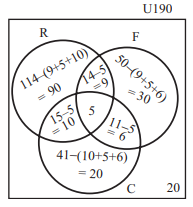The number of students who liked any one of the music = 90 + 9 + 30 + 10 + 5 + 6 + 20

= 170

(a) The number of students who didn’t like any 3 types of music = 190 – 170 = 20

(b) Number of students who liked any two types ony = 9 + 6 + 10 = 25

(c) Number of students who liked folk music but not rock music = 30 + 6 = 36

32. A function f : [-3, 7) → R is defined as follows: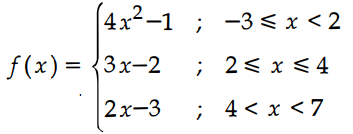Find:

(i) f(-2) – f(4)

(ii) [f(3) + f(-1)]/ [2f(6) – f(1)]

Solution:

(i) f(-2) = 4x2 – 1 = 4(-2)2 – 1 = 16 – 1 = 15

f(4) = 3x – 2 = 3(4) – 2 = 12 – 2 = 10

f(-2) – f(4) = 15 – 10 = 5

(ii) f(3) = 3x – 2 = 3(3) – 2 = 9 – 2 = 7

f(-1) = 4x2 – 1 = 4(-1)2 – 1 = 4 – 1 = 3

f(6) = 2x – 3 = 2(6) – 3 = 12 – 3 = 9

f(1) = 4x2 – 1 = 4(1)2 – 1 = 4 – 1 = 3

[f(3) + f(-1)]/ [2f(6) – f(1)] = [(7 + 3)/ (2 × 9 – 3)]

= 10/(18 – 3)

= 10/15

= 2/3

33. Find the sum to n terms of the series 7 + 77 + 777 + …

Solution:

Given,

7 + 77 + 777 +…

Sum of first n terms

Sn = 7 + 77 + 777 + ….. + (n terms)

Sn = 7(1 + 11 + 111 + …. + n terms)

= (7/9) [9 + 99 + 999 + … + n terms]

= (7/9) [(10 – 1) + (100 – 1) + (1000 – 1) + …. + (10n – 1)]

= (7/9) [(10 + 102 + 103 + … + 10n) – (1 + 1 + 1 + … + n terms)]

= (7/9) {[10(10n – 1)/ (10 – 1)] – n}

= (7/9) {[10(10n – 1)/9] – n}

= (70/81) (10n – 1) – 7n/9

34. If 7 times the 7th term of an Arithmetic Progression is equal to 11 times its 11th term, show that its 18th term is zero. Can you find the first term and the common difference? Justify your answer.

Solution:

Let a be the first term and d be the common difference an AP.

Given,

7a7 = 11a11

7(a + 6d) = 11(a + 10d)

7a + 42d = 11a + 110d

⇒ 11a + 110d – 7a – 42d = 0

⇒ 4a + 68d = 0

⇒ 4(a + 17) = 0

⇒ a + 17 = 0

⇒ a18 = 0

It is not possible here to find both the first term and common difference. Since only one equation obtained from the given data.

35. Factorise: x3 – 5x2 – 2x + 24

Solution:

Let the given polynomial be p(x) = x3 – 5x2 – 2x + 24

Let us substitute various values of x until we get p(x) = 0.

p(0) = (0)3 – 5(0)2 – 2(0) + 24 = 24 ≠ 0

p(1) = (1)3 – 5(1)2 – 2(1) + 24 = 1 – 5 – 2 + 24 = 18 ≠ =

p(-2) = (-2)3 – 5(-2)2 – 2(-2) + 24 = -8 – 10 + 4 + 24 = 0

Therefore, x + 2 is a factor of p(x).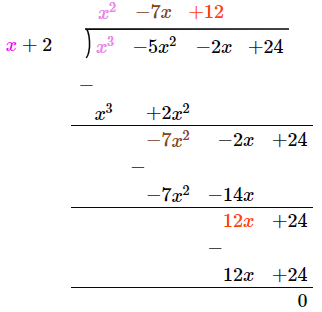p(x) = (x + 2)(x2 – 7x + 12)

= (x + 2)[x2 – 3x – 4x + 12]

= (x + 2) [x(x – 3) – 4(x – 3)]

= (x + 2)(x – 3)(x – 4)

Hence, the factors of the given polynomial are (x + 2), (x – 3) and (x – 4).

36. Simplify: [1/(x2 + 3x + 2)] + [1/(x2 + 5x + 6)] – [2/(x2 + 4x + 3)]

Solution:

x2 + 3x + 2 = x2 + 2x + x + 2 = (x + 1)(x + 2)

x2 + 5x + 6 = x2 + 2x + 3x + 6 = (x + 2)(x + 3)

x2 + 4x + 3 = x2 + 3x + x + 3 = (x + 1)(x + 3)

Now,

[1/(x2 + 3x + 2)] + [1/(x2 + 5x + 6)] – [2/(x2 + 4x + 3)]

= [1/ (x + 1)(x + 2)] + [1/ (x + 2)(x + 3)] – [2/ (x + 1)(x + 3)]

= [(x + 3) + (x + 1) – 2(x + 2)]/ [(x + 1)(x + 2)(x + 3)]

= [2x + 4 – 2x – 4]/ [(x + 1)(x + 2)(x + 3)]

= 0

37.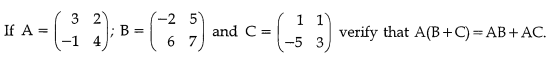Solution: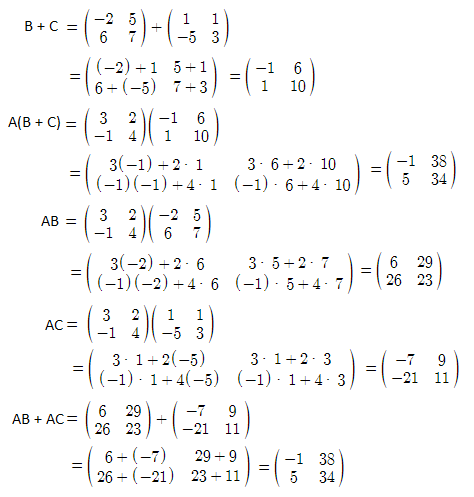Therefore, A(B + C) = AB + AC

38. Find the area of the quadrilateral whose vertices are (-4, 5), (0, 7), (5, -5) and (-4, -2).

Solution:

Given, vertices of the quadrilateral are (-4, 5), (0, 7), (5, -5) and (-4, -2).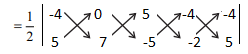= 1/2 |(-28 – 0 – 10 – 20) – (0 + 35 + 20 + 8)|

= (1/2) |-58 – 63]|

= (1/2) × 121

= 60.5 sq.units

39. State and prove Thales theorem.

Solution:

Statement:

If a line is drawn parallel to one side of a triangle to intersect the other two sides in distinct points, the other two sides are divided in the same ratio.

Proof:

In triangle ABC, a line parallel to side BC intersects other two sides namely AB and AC at D and E respectively.

Join BE and CD.

Also, draw DM ⊥ AC and EN ⊥ AB.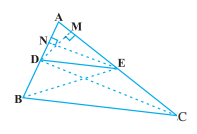Similarly,

ar(∆BDE) = 1/2 (DB × EN)

ar(∆ADE) = 1/2 (AE × DM)

ar(∆DEC) = 1/2 (EC × DM)

Now,

ar(∆ADE)/ar(∆DEC) = [1/2 (AE × DM)]/ [1/2 (EC × DM)] = AE/EC ….(i)

Triangle BDE and DEC are on the same base DE and between the same parallels.

Therefore, ar(∆BDE) = ar(∆DEC) ….(iii)

From (i), (ii) and (iii),

Hence proved.

BC and DE.

40. A person in a helicopter flying at a height of 700 m, observes two objects lying opposite to each other on either banks of a river. The angles of depression of the objects are 30° and 45°; find the width of the river. (√3 = 1.732)

Solution:

Let A be the position of flight.

B and C be the positions of two objects.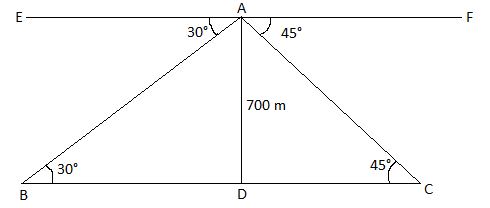1 = 700/CD

⇒ CD = 700 m

1/√3 = 700/BD

⇒ BD = 700√3 m

Width of the river = BD + CD = 700√3 + 700

= 700(√3 + 1)

= 700(1.732 + 1)

= 700 × 2.732

= 1912.40 m

41. The radii of two circular ends of a frustum shaped bucket are 15 cm and 8 cm. If its depth is 63 cm, find the capacity of the bucket in litres. (Take π = 22/7)

Solution:

Given,

The radii of two circular ends of a frustum shaped bucket are 15 cm and 8 cm.

R = 15 cm and r = 8 cm

Depth = h = 63 cm

Volume of frustum shaped bucket = = (1/3)πh[R2 + r2 + Rr]

= (1/3) × (22/7) × 63 × [152 + 82 + (15)(8)]

= (66) × [225 + 64 + 120]

= 66 × 409

= 26994 cm3

= 26.994 lit

Hence, the capacity of the bucket is 26.994 lit.

42. A circus tent is to be erected in the form of a cone surmounted on a cylinder. The total height of the tent is 49 m. Diameter of the base is 42 m and the height of the cylinder is 21 m. Find the cost of canvas needed to make the tent, if the cost of canvas is Rs. 12.50/m2. (Take π = 22/7)

Solution:

Given,

Diameter of cylinder = 42 m

Radius = r = 21 m

Height of cylinder = h = 21 m

Curved surface area of cylinder = 2πrh

= 2 × (22/7) × 21 × 21

= 44 × 62

= 2772 m2

Radius of the cone = R = 21 m (given)

Height = H = 28 m

Slant height = l = √(R2 + H2)

= √(212 + 282)

= √(441 + 784)

= √1225

= 35 m

Curved surface area of cone = πRl

= (22/7) × 21 × 35

= 2310 m2

Total surface area of circus tent = 2772 + 2310 = 5082 m2

Cost of canvas 1 m2 = Rs. 12.50

Total cost of canvas = 5082 × Rs. 12.50 = Rs. 63525

43. Calculate the standard deviation of the following data.

 x 3 8 13 18 23 f 7 10 15 10 8

Solution:

 x f d = x – 13 d2 fd2 3 7 -10 100 700 8 10 -5 25 250 13 15 0 0 0 18 10 5 25 250 23 8 10 100 800 ∑d = 50 ∑d2 = 2000

σ = √(Σfd²/Σf)

= √(2000/50)

= √40

= 6.325

Therefore, the standard deviation is 6.325.

44. A box contains 4 white balls, 6 red balls, 7 black balls and 3 blue balls. One ball is drawn at random from the bag. Find the probability that the ball drawn is :

(i) ·neither white nor black

(ii) red or white, and

(iii) either white or red or black or blue

Solution:

Given,

4 white balls, 6 red balls, 7 black balls and 3 blue balls

n(S) = 4 + 6 + 7 + 3 = 20

(i) Number of balls other than white and black = 6 (red) + 3 (blue) = 9

P(neither white nor black) = 9/20

(ii) Probability of getting red ball = P(R) = 6/20

Probability of getting white ball = P(W) = 4/20

P(red or white ball) = P(R) + P(W)

= (6/20) + (4/20)

= 10/20

= ½

(iii) Probability of getting black ball = 7/20

Probability of getting blue ball = 3/20

P(either white or red or black or blue) = (6/20) + (4/20) + (7/20) + (3/20)

= 20/20

= 1

45. (a) If the equation (1 + m2)x2 + 2mcx + c2 – a2 = 0 has equal roots, then prove that c2 = a2(1 + m2)

Solution:

Given that the equation (1 + m2)x2 + 2mcx + c2 – a2 = 0 has equal roots.

Therefore, discriminant = 0

⇒ (2mc)2 – 4(1 + m2)(c2 – a2) = 0

⇒ 4m2c2 – 4[c2 – a2 + m2c2 – m2a2] = 0

⇒ 4m2c2 – 4c2 + 4a2 – 4m2c2 + 4m2a2 = 0

⇒ -4c2 + 4a2(1 + m2) = 0

⇒ 4c2 = 4a2(1 + m2)

⇒ c2 = a2(1 + m2)

Hence proved.

OR

(b) Find the equation of the straight line segment whose endpoints are the points of intersection of the straight lines 2x – 3y + 4 = 0, x – 2y + 3 = 0 and the midpoint of the line joining the points (3, -2) and (-5, 8).

Solution:

Given equations of lines are:

2x – 3y + 4 = 0….(i)

x – 2y + 3 = 0….(ii)

(ii) × 2 – (i),

2x – 4y + 6 – (2x – 3y + 4) = 0

-y + 2 = 0

y = 2

Substituting y = 2 in (ii)

x – 2(2) + 3 = 0

x – 4 + 3 = 0

x = 1

Therefore, the point of intersection of lines = A(1, 2)

Midpoint of the line joining the points (3, -2) and (-5, 8)= B = [(3 – 5)/2, (-2 + 8)/2]

= (-2/2, 6/2)

= (-1, 3)

Equation of the line segment joining A and B is

(y – y1)/ (y2 – y1) = (x – x1)/ (x2 – x1)

(y – 2)/ (3 – 2) = (x – 1)/ (-1 – 1)

(y – 2)/1 = (x – 1)/ (-2)

(-2)(y – 2) = x – 1

-2y + 4 = x – 1

⇒ x + 2y – 1 – 4 = 0

⇒ x + 2y – 5 = 0

SECTION – IV

46. (a) Draw the two tangents from a point which is 9 cm away from the centre-of a circle of radius 3 cm. Also, measure the lengths of the tangents.

Solution: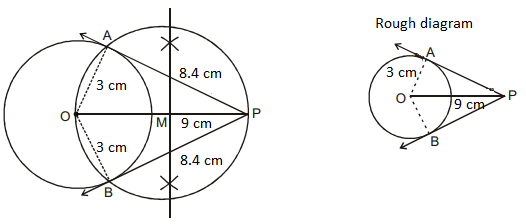Length of the tangents = 8.4 cm

OR

(b) Construct a cyclic quadrilateral PQRS given PQ = 5 cm, QR = 4 cm, ∠QPR = 35° and ∠PRS = 70°.

Solution: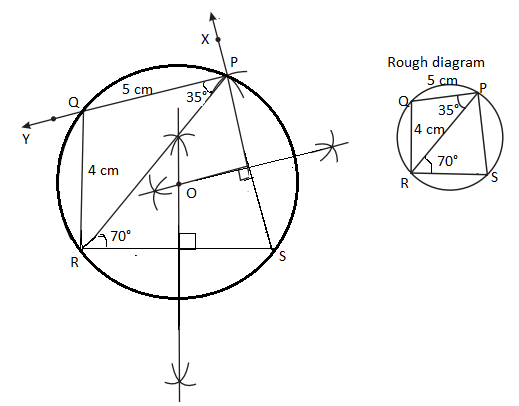47. (a) Draw the graph of y = x2 + 2x – 3 and hence find the roots of x2 – x – 6 = 0.

Solution:

Given,

y = x2 + 2x – 3

 x -3 -2 -1 0 1 2 3 y 0 -3 -4 -3 0 5 12

Now, subtracting x2 – x – 6 = 0 from y = x2 + 2x – 3,

y – 0 = x2 + 2x – 3 – (x2 – x – 6)

y = 3x + 3

 x -2 -1 0 1 2 3 y -3 0 3 6 9 12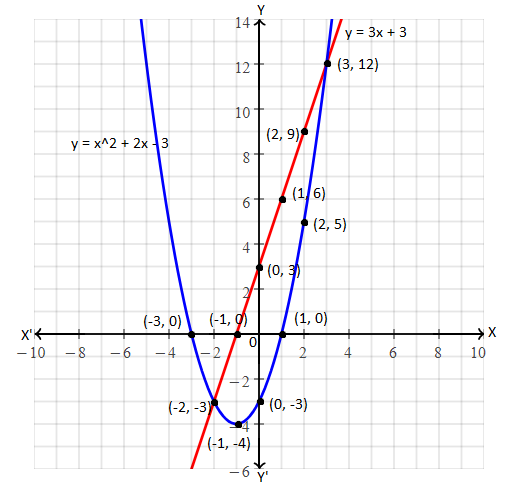The graph of given equations intersect each other at (-2, -3) and (3, 12).

Hence, the solution set is (-2, -3) and (3, 12).

OR

(b)

 Number of workers 3 4 6 8 9 16 Number of days 96 72 48 36 32 18

Draw the graph for the data given in the table. Hence find the number of days taken by 12 workers to complete the work.

Solution: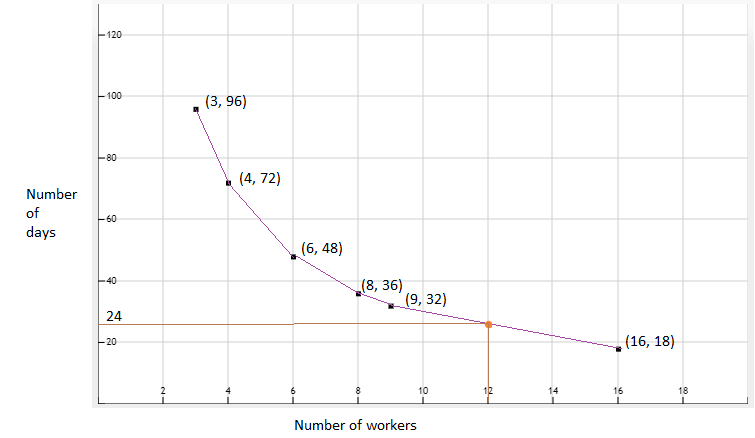Hence, the number of days taken by 12 workers to complete the work is 24.